# RS Aggarwal Solutions for Class 8 Maths Chapter 2 - Exponents

RS Aggarwal Solutions For Class 8 Maths Chapter 2 Exponents are provided here. You can download the pdf of these Solutions from the given links. RS Aggarwal Solutions helps students to obtain a good score in the examinations, it also provides extensive knowledge about the subject, as Class 8 is a critical stage in their academic career. Therefore, we at BYJU’S provide answers to all questions uniquely and briefly. RS Aggarwal Solutions For Class 8 Maths Chapter 2 Exponents also provides the foundation to higher studies as well as helps the students in effectively understanding the concepts.

## Download pdf of RS Aggarwal Solutions For Class 8 Maths Chapter 2 Exponents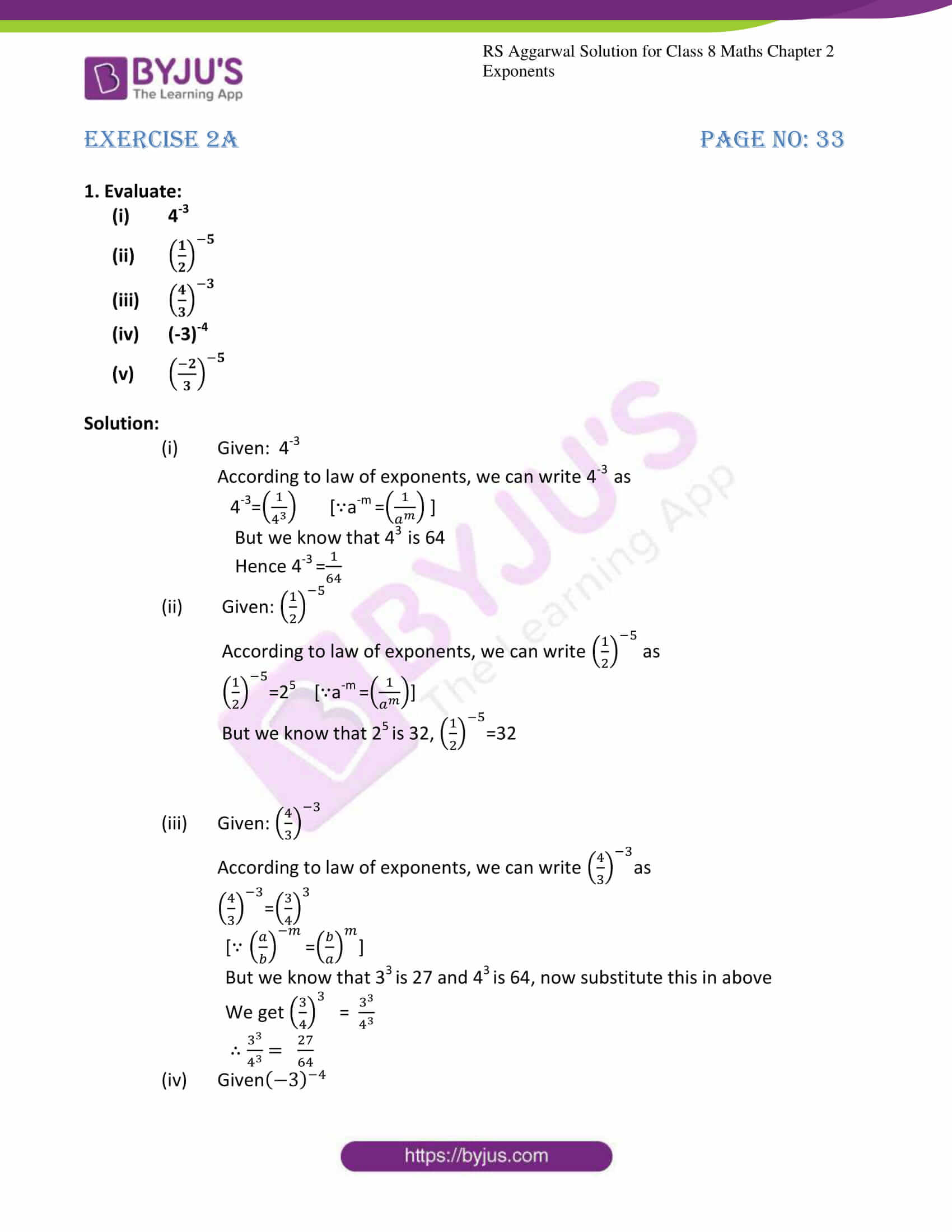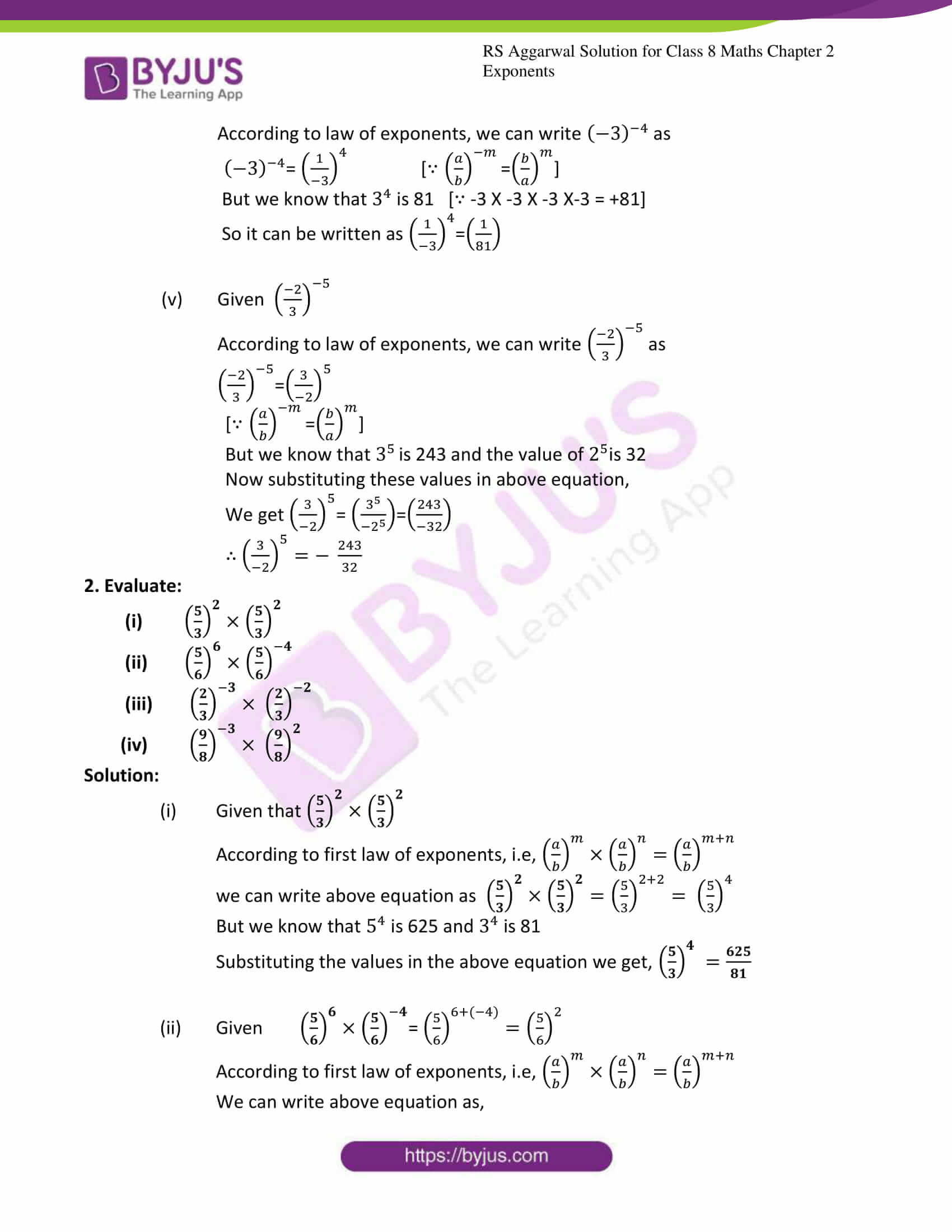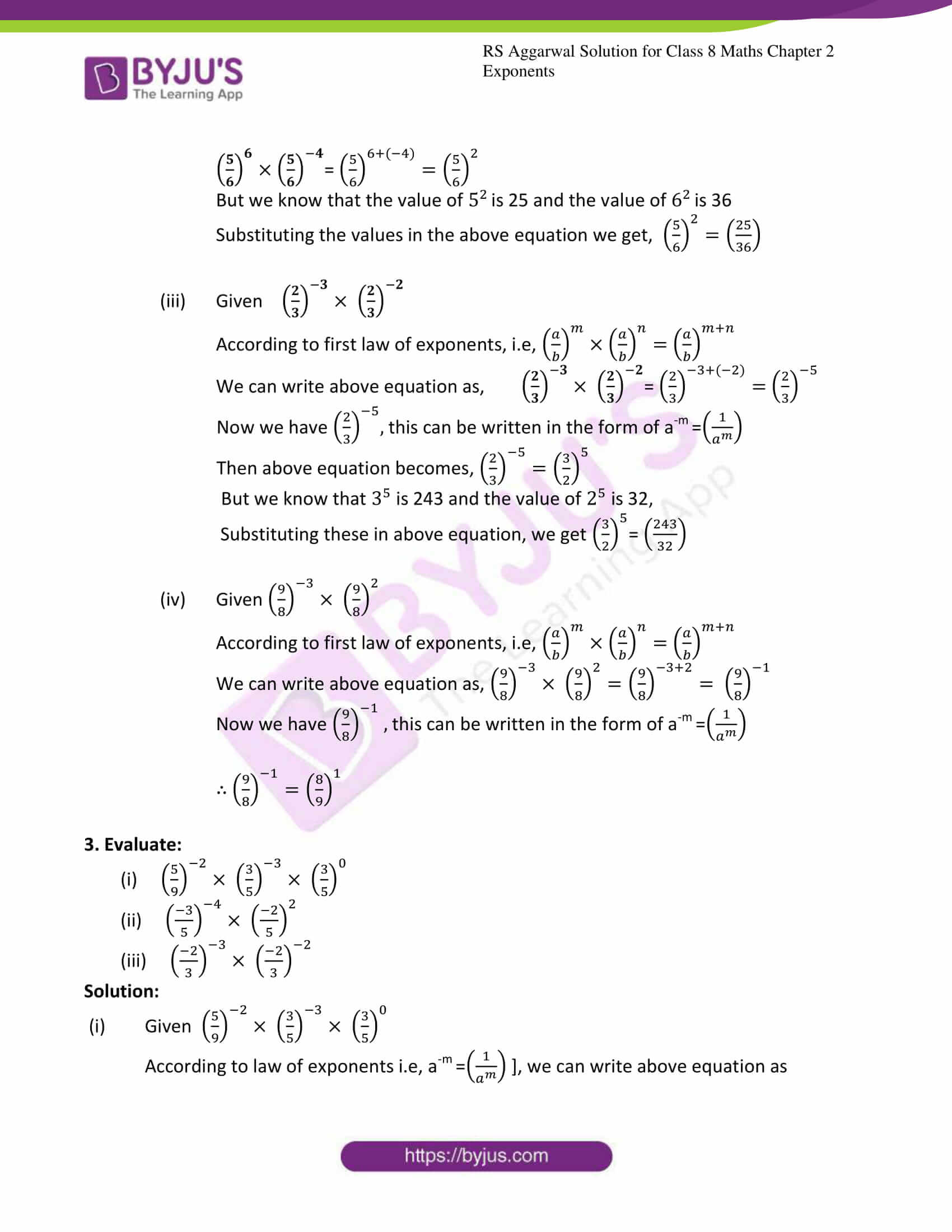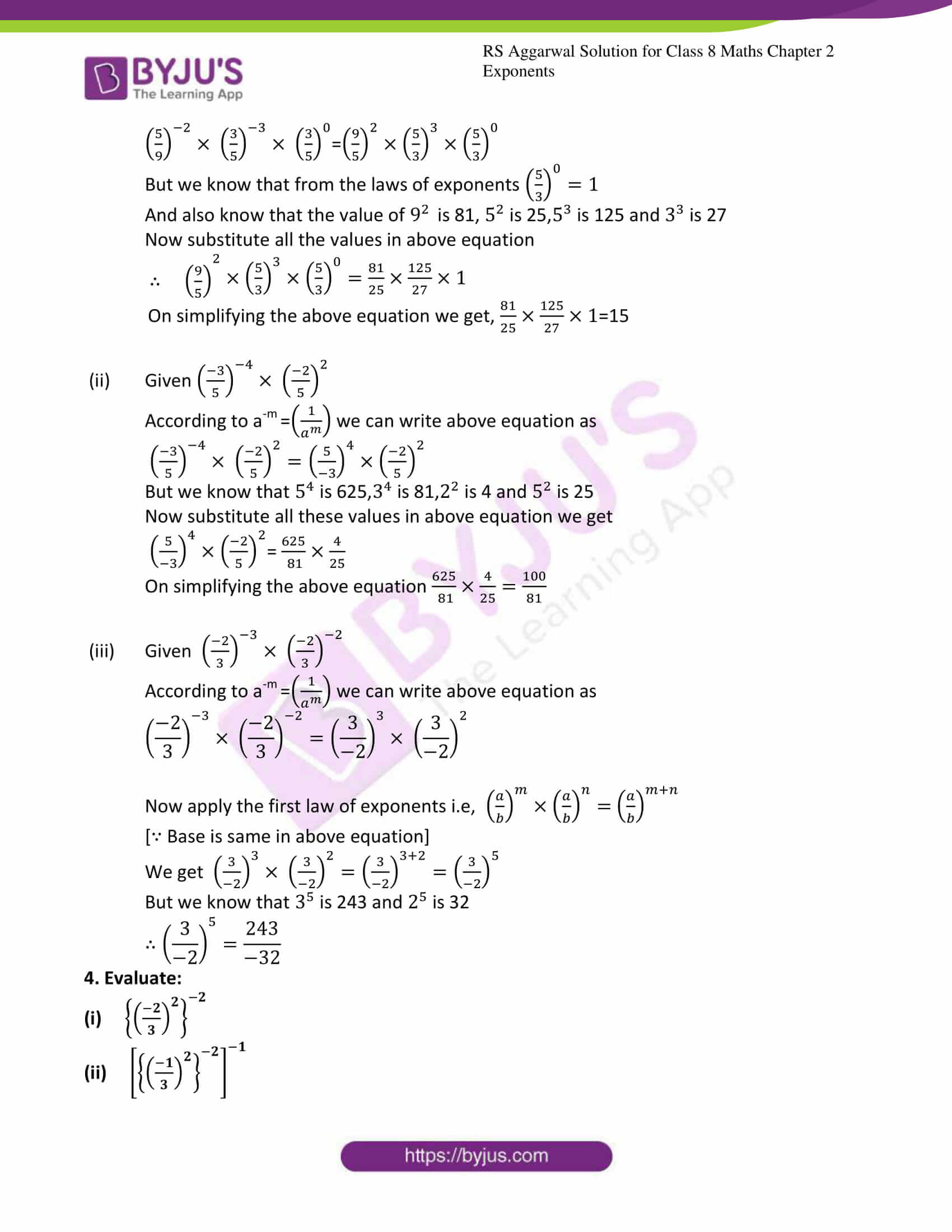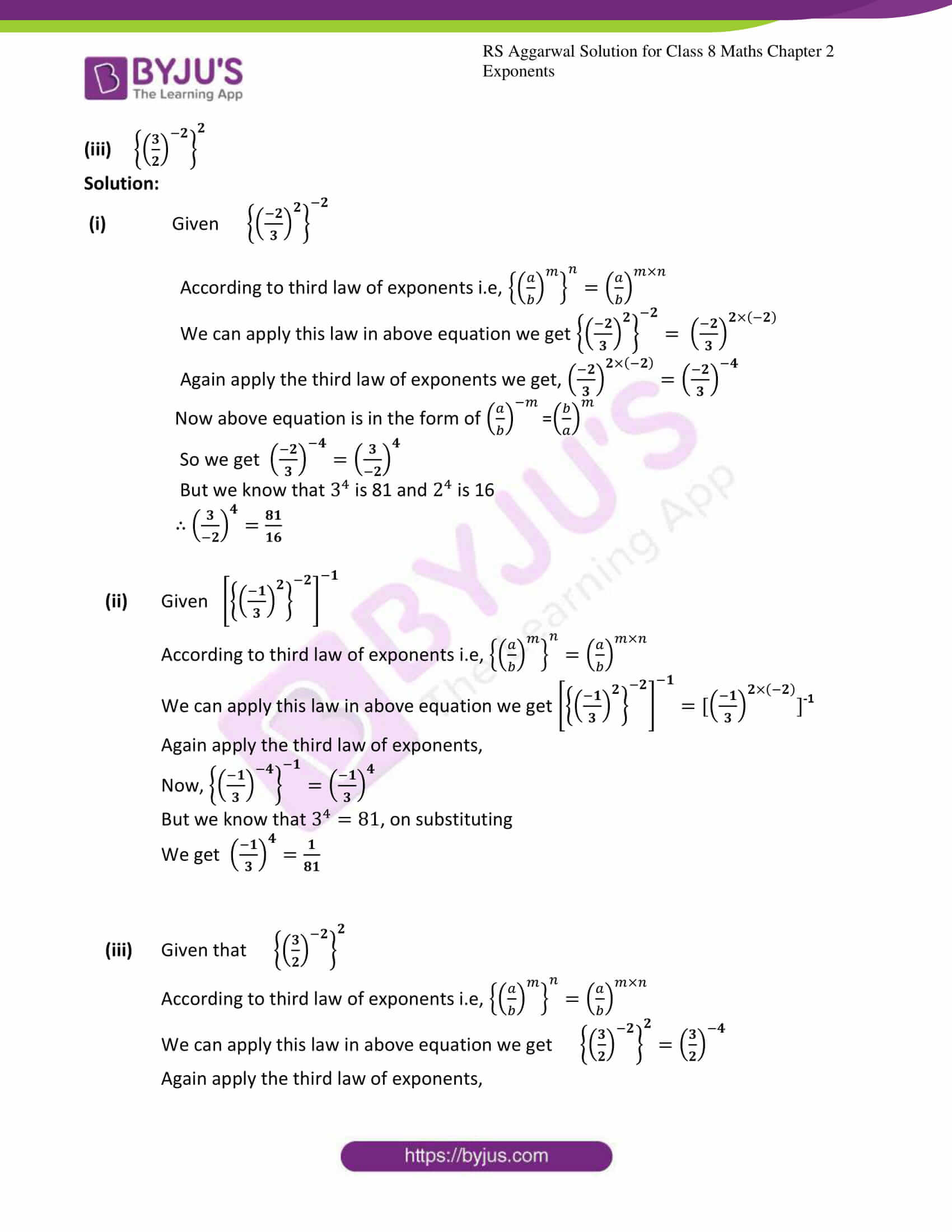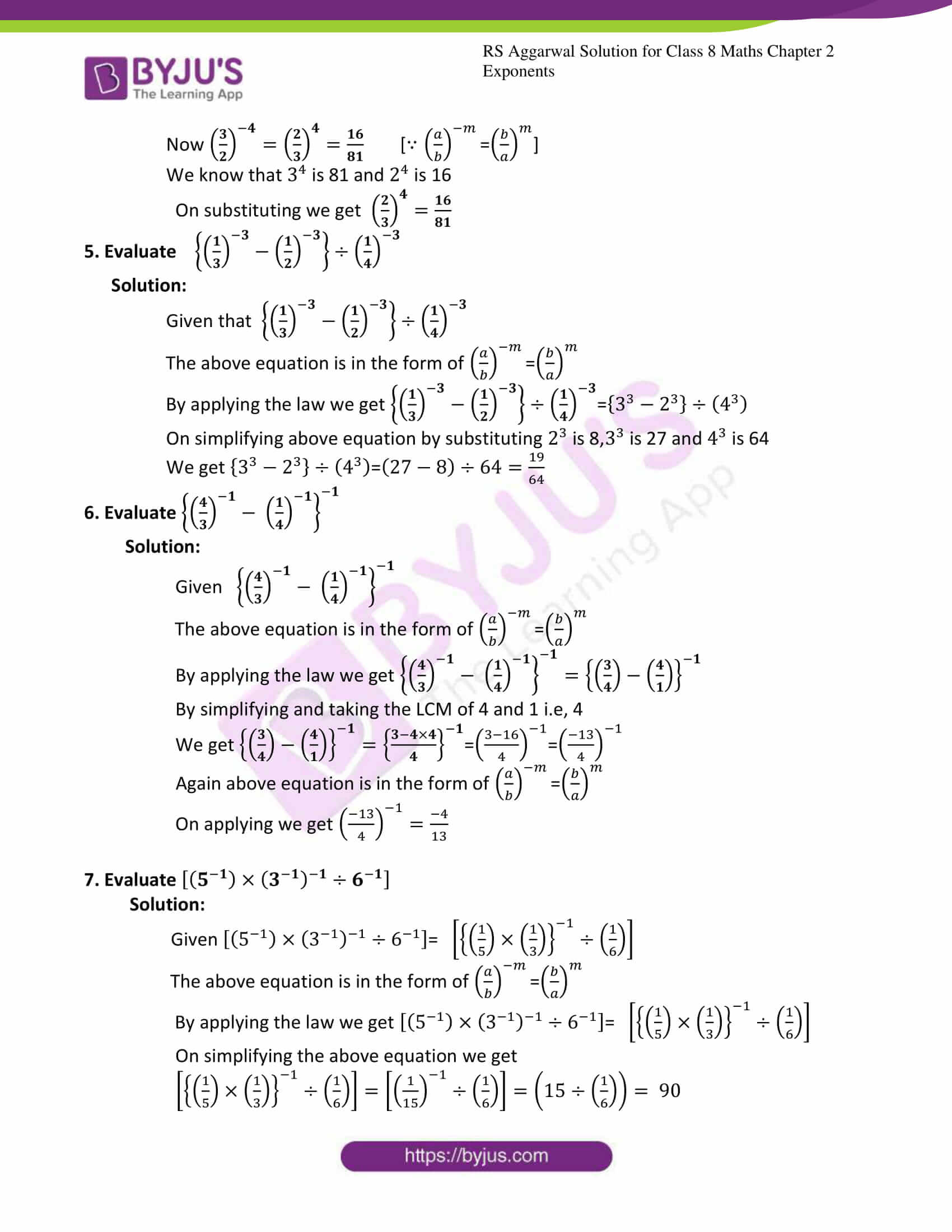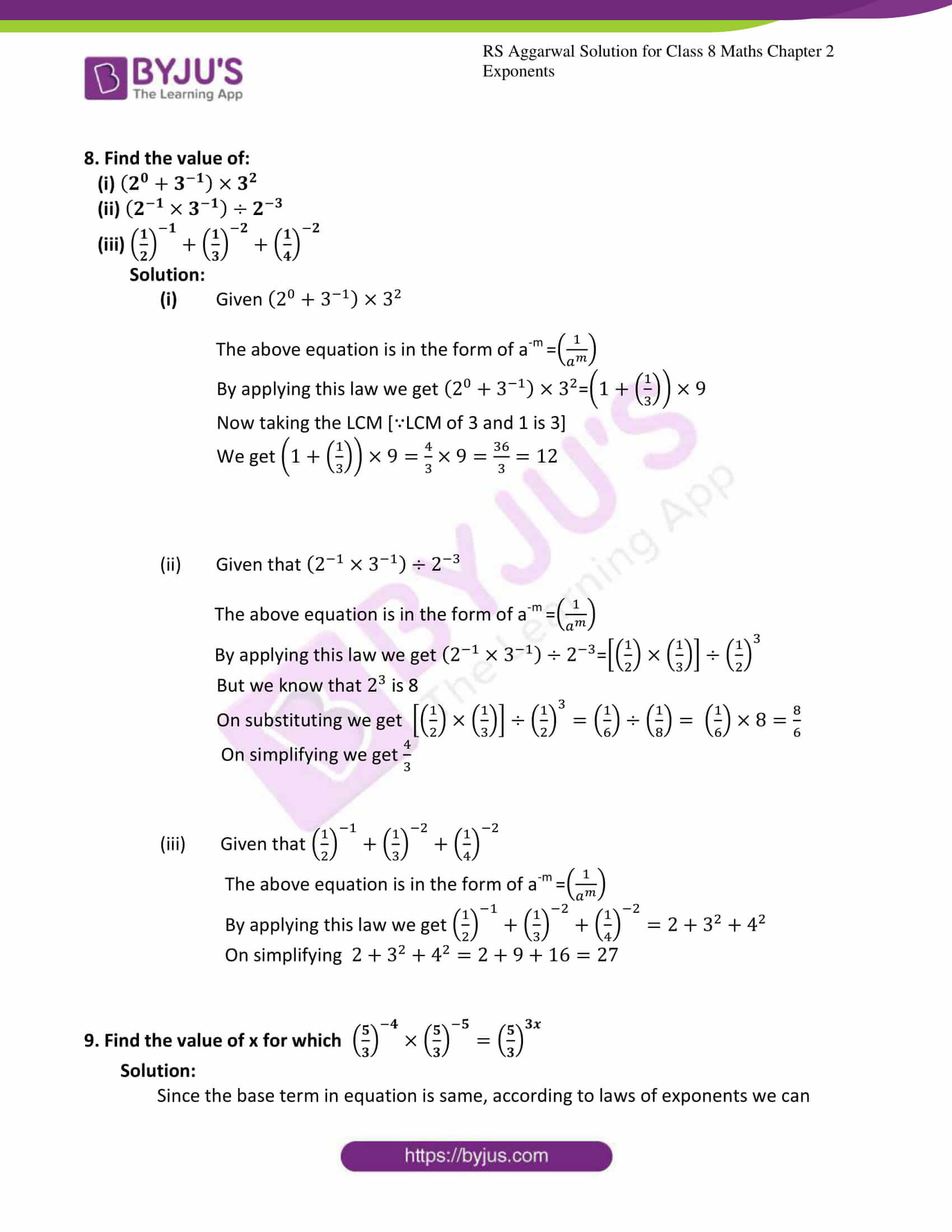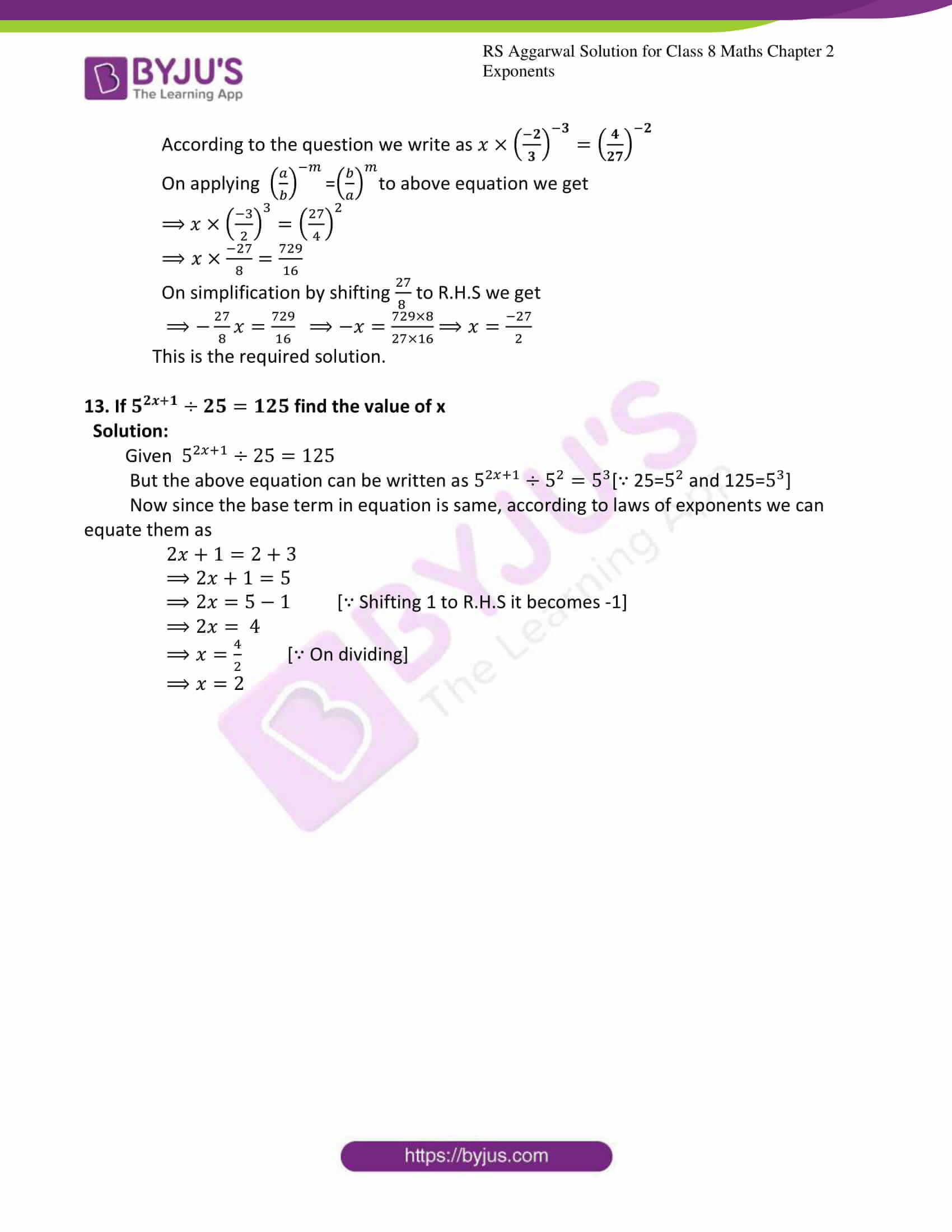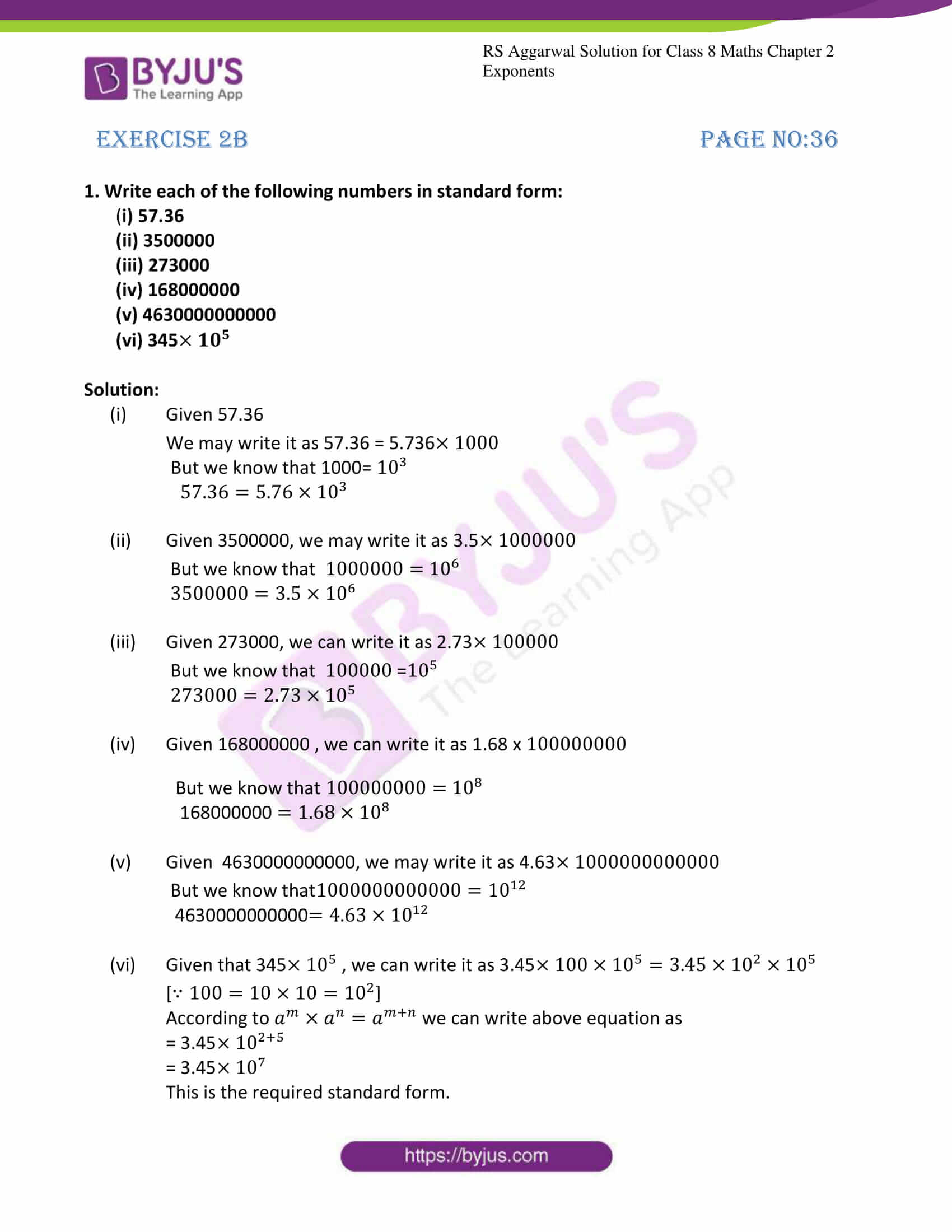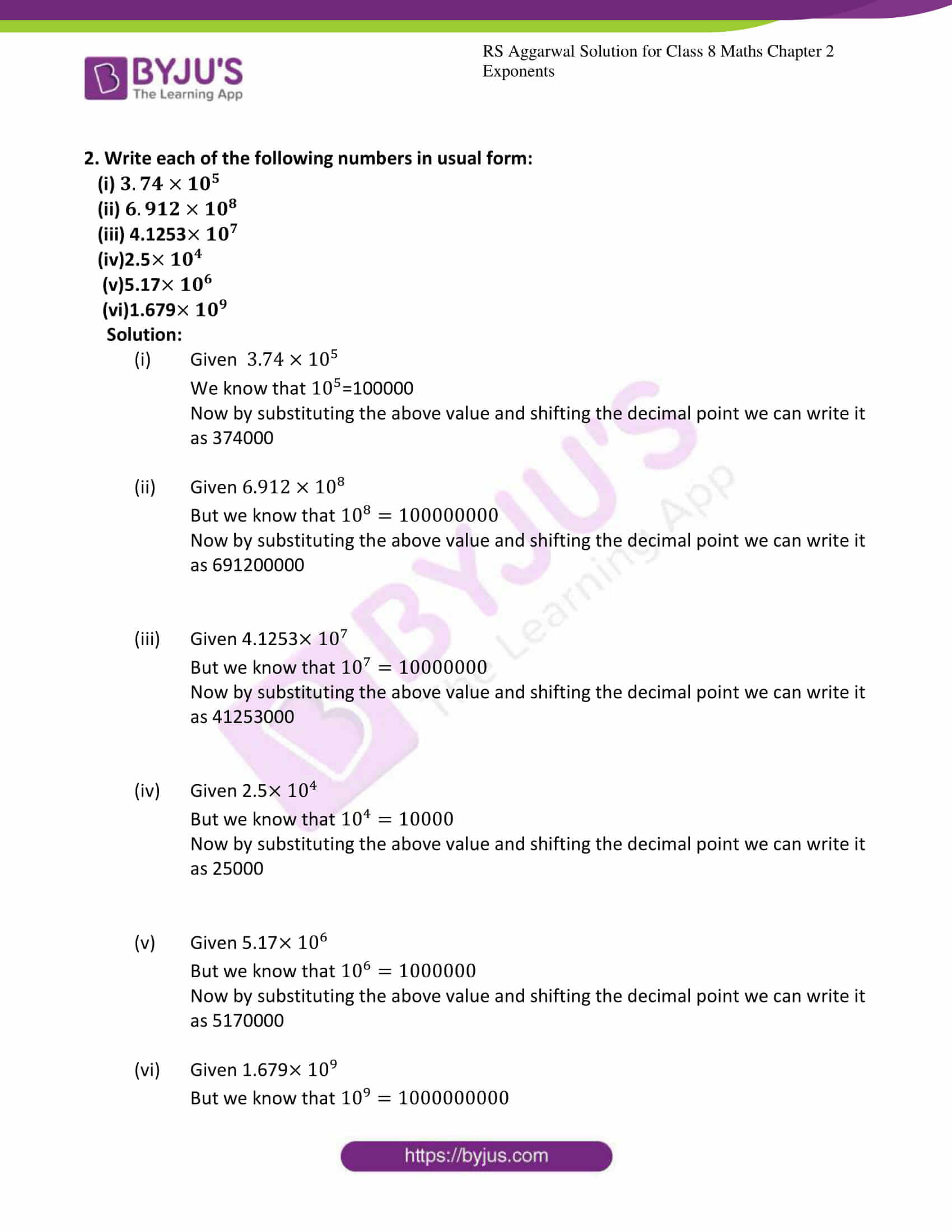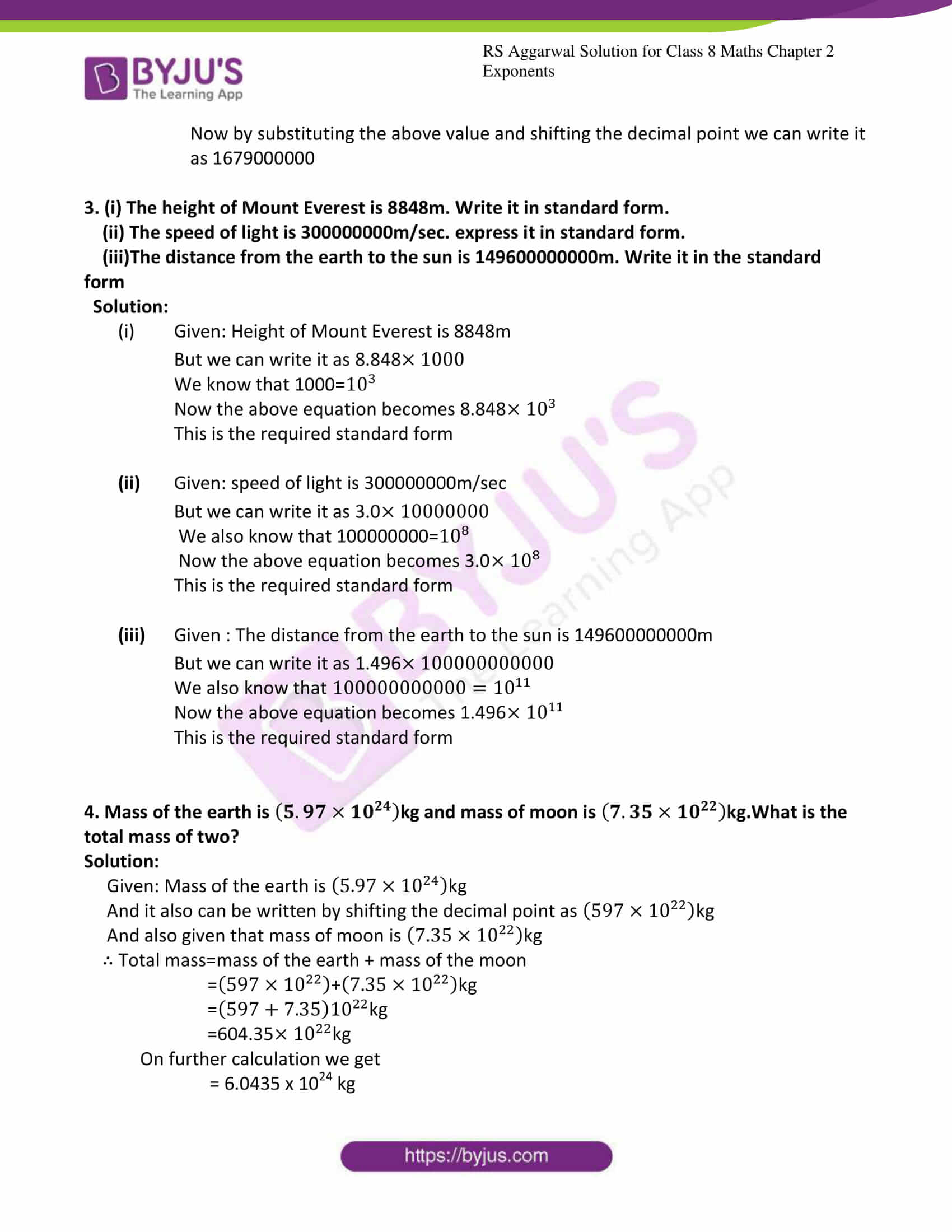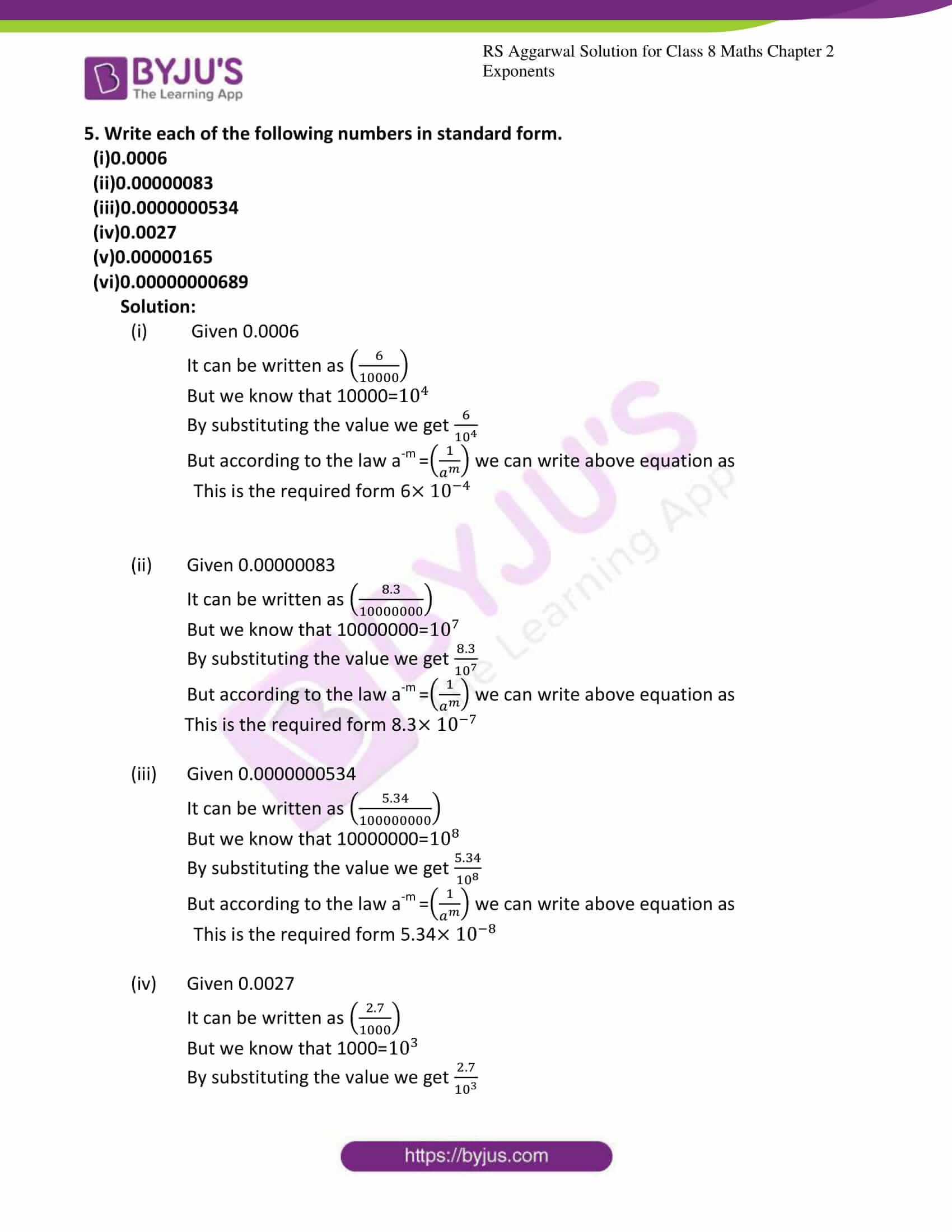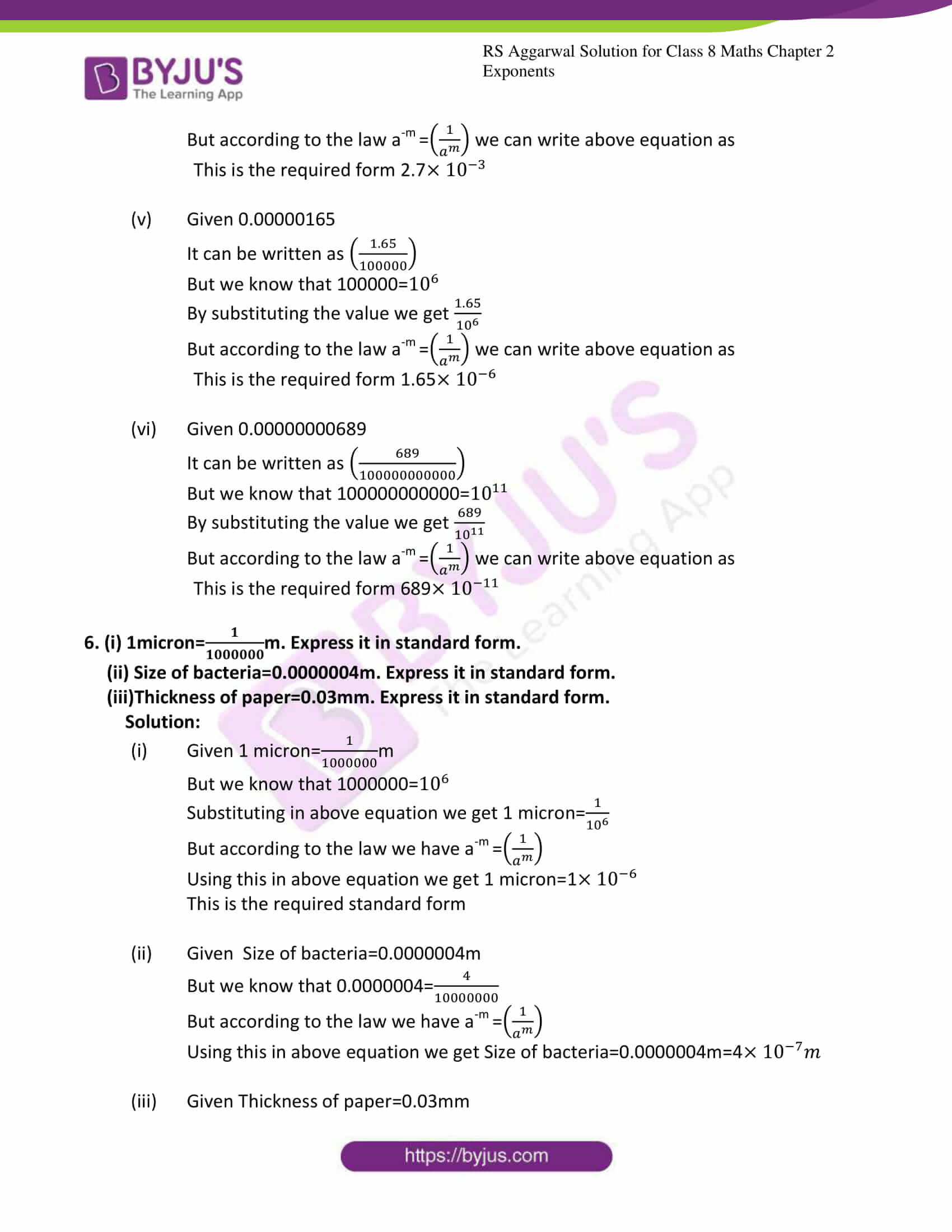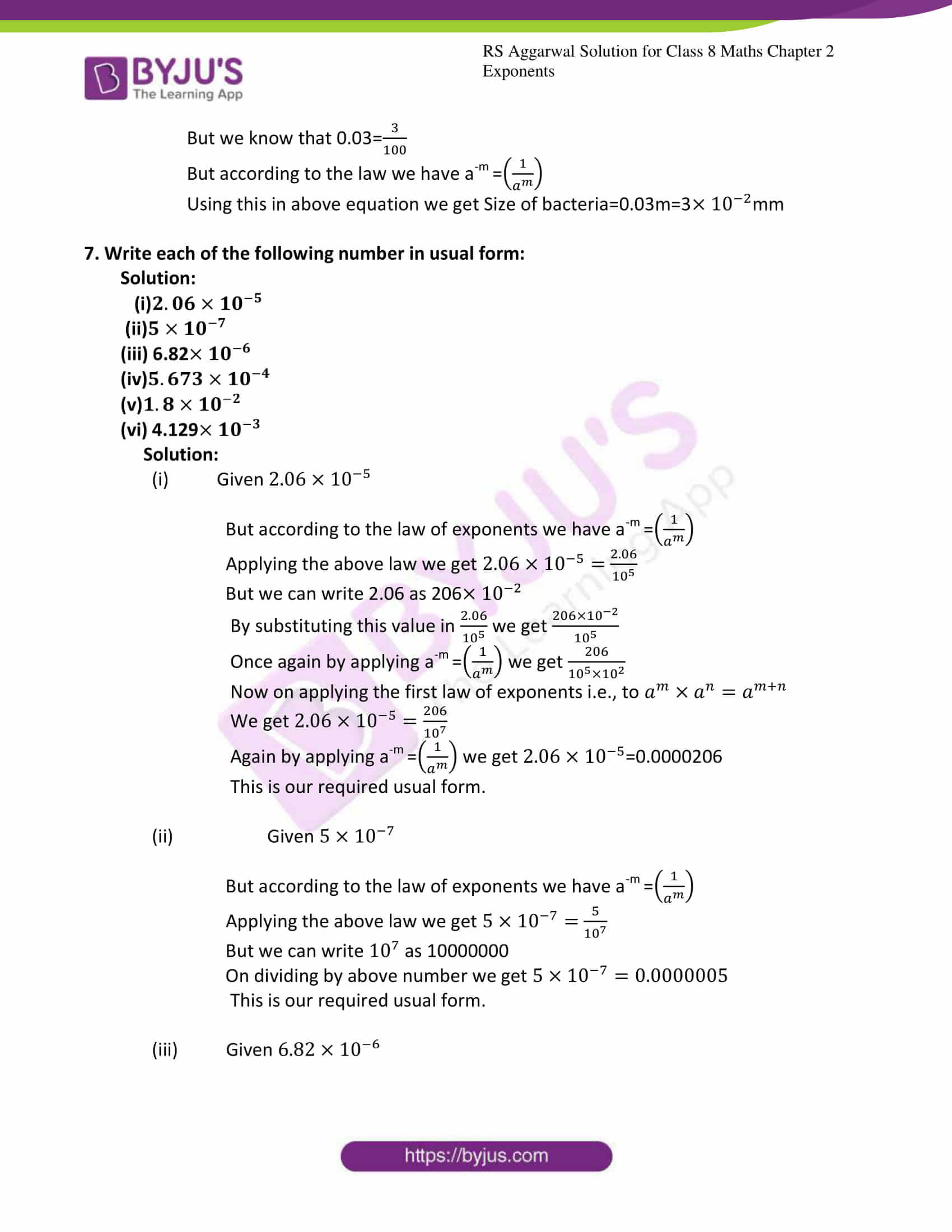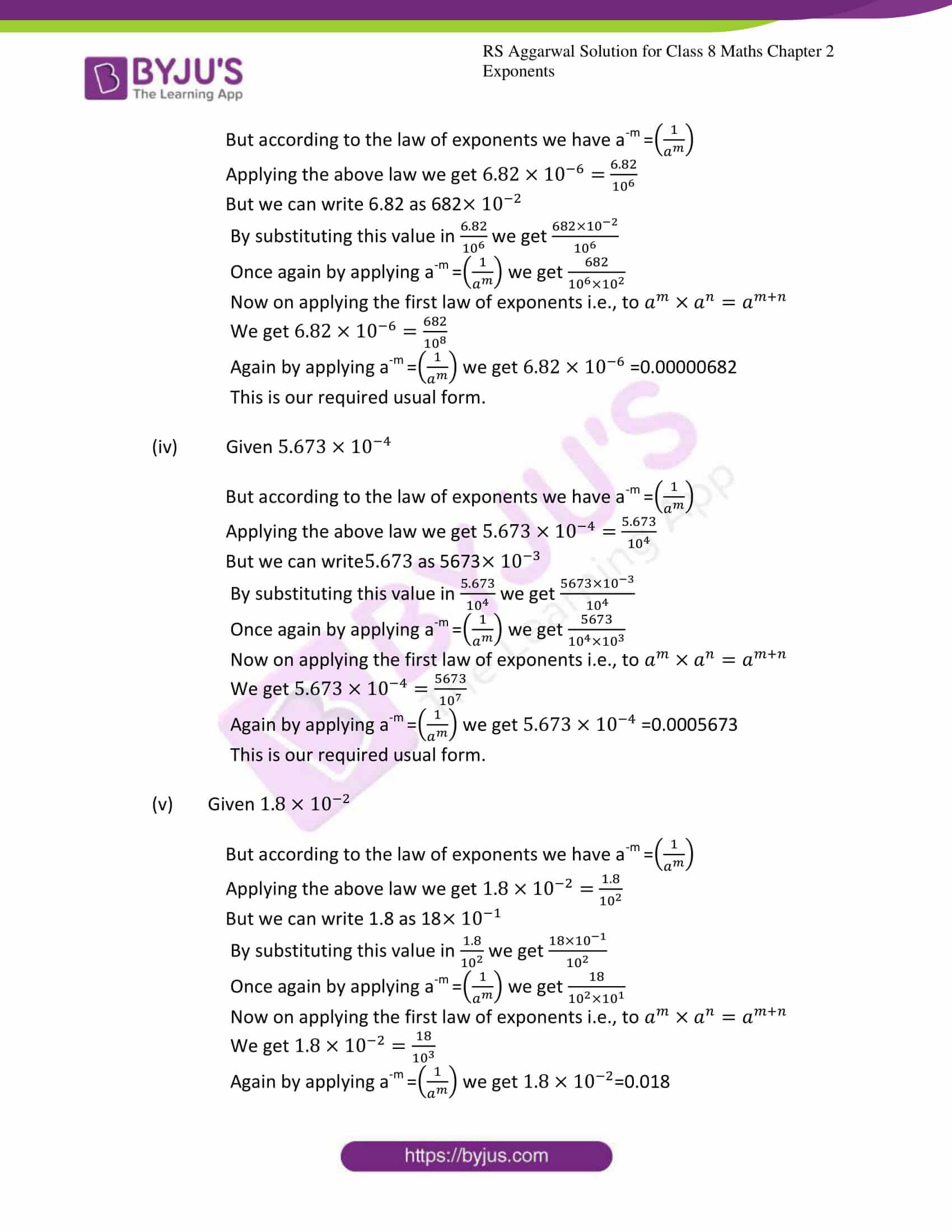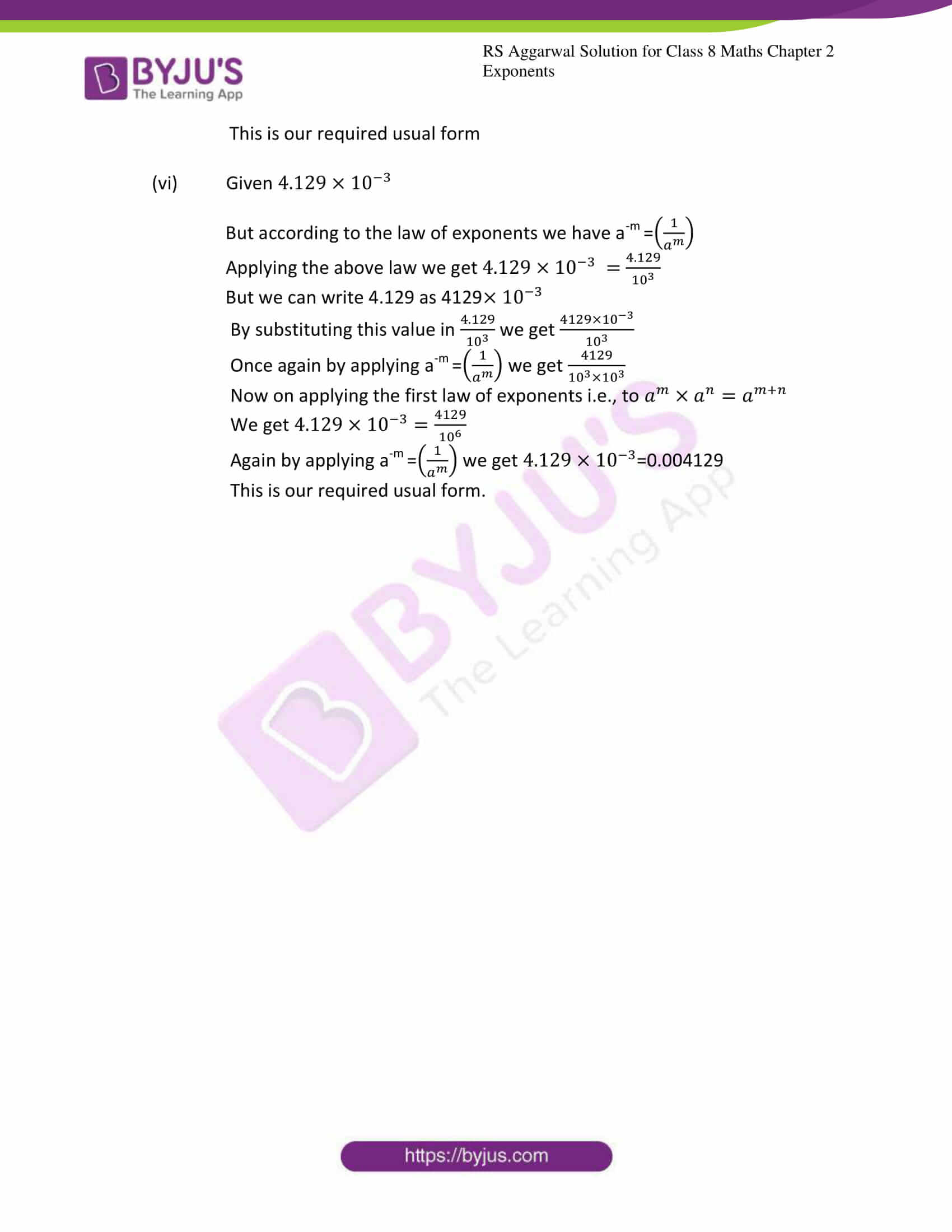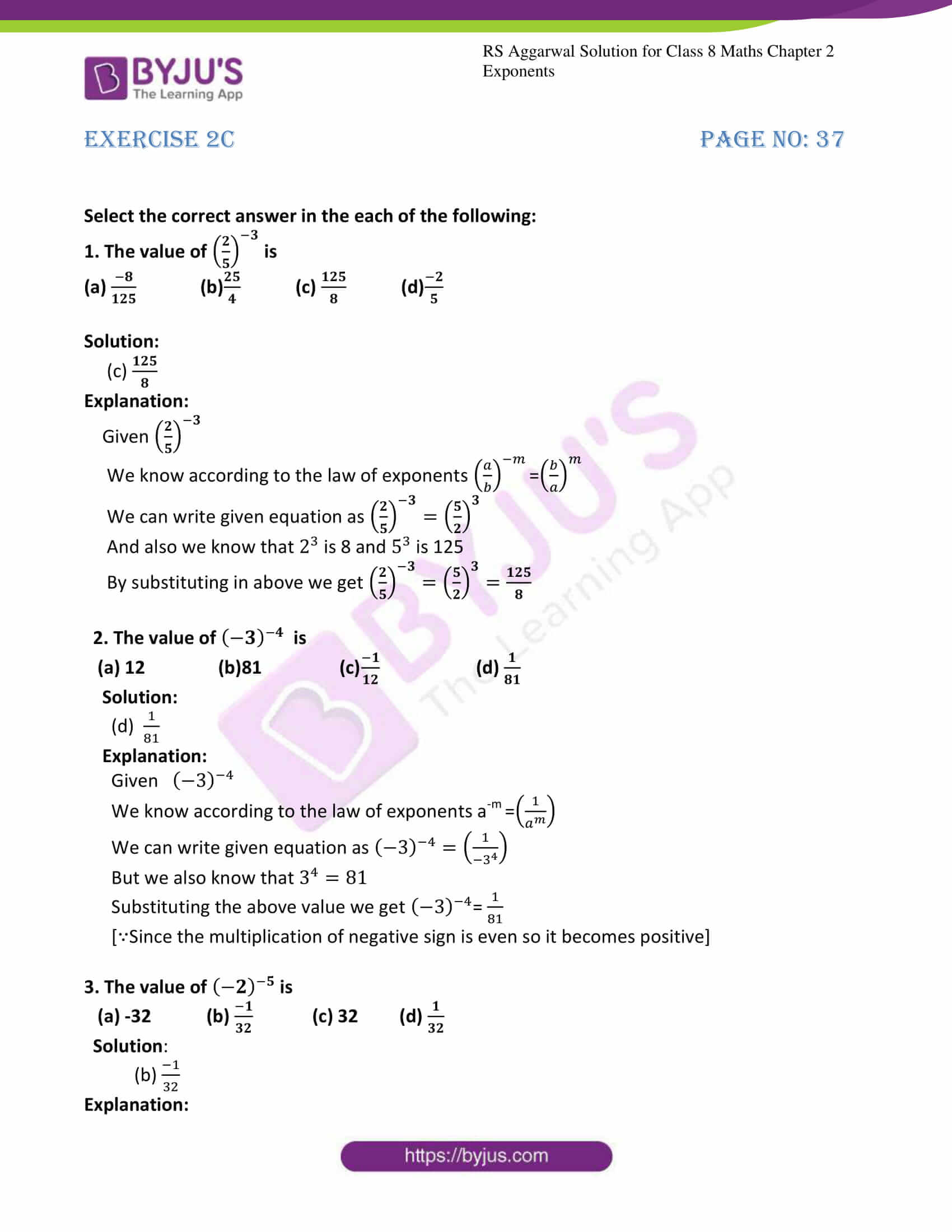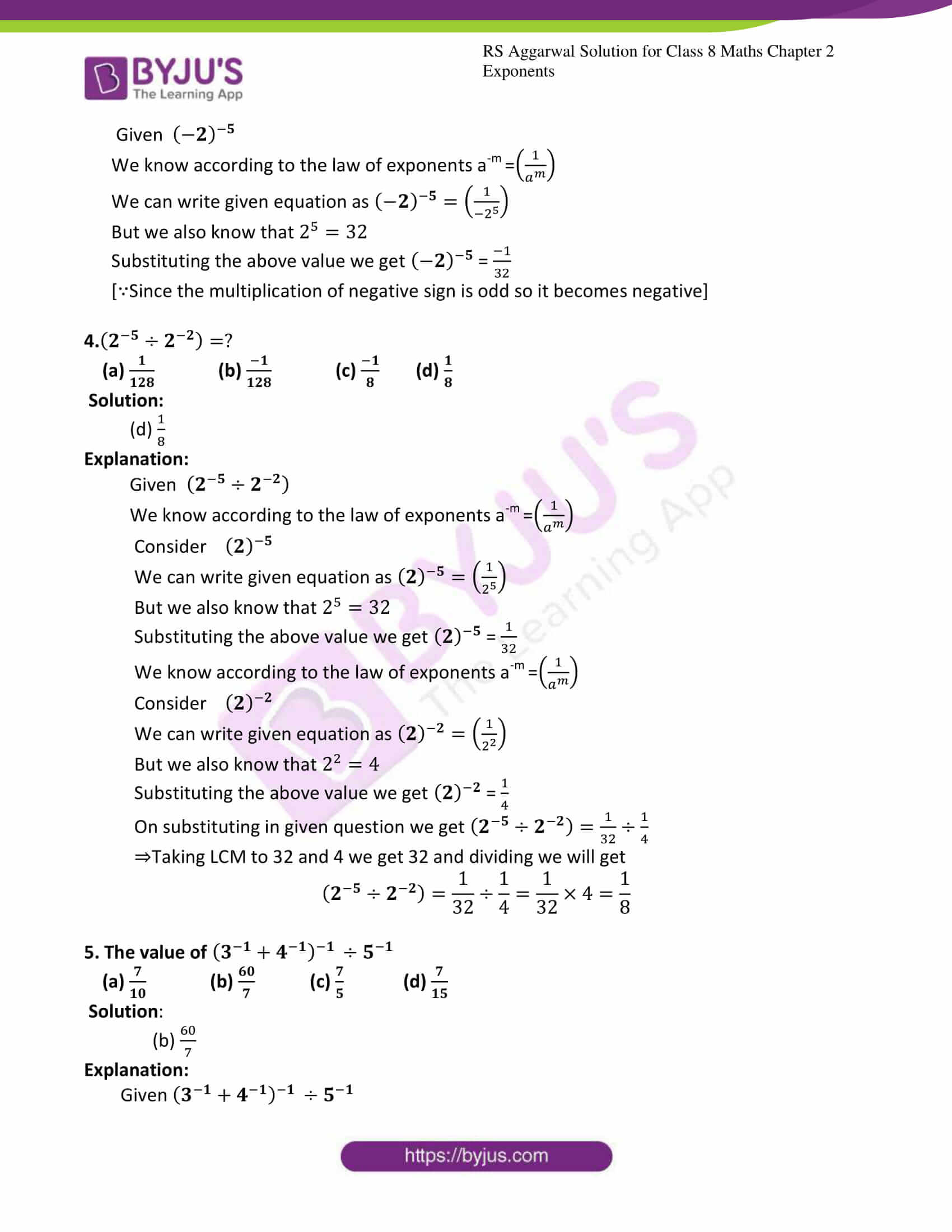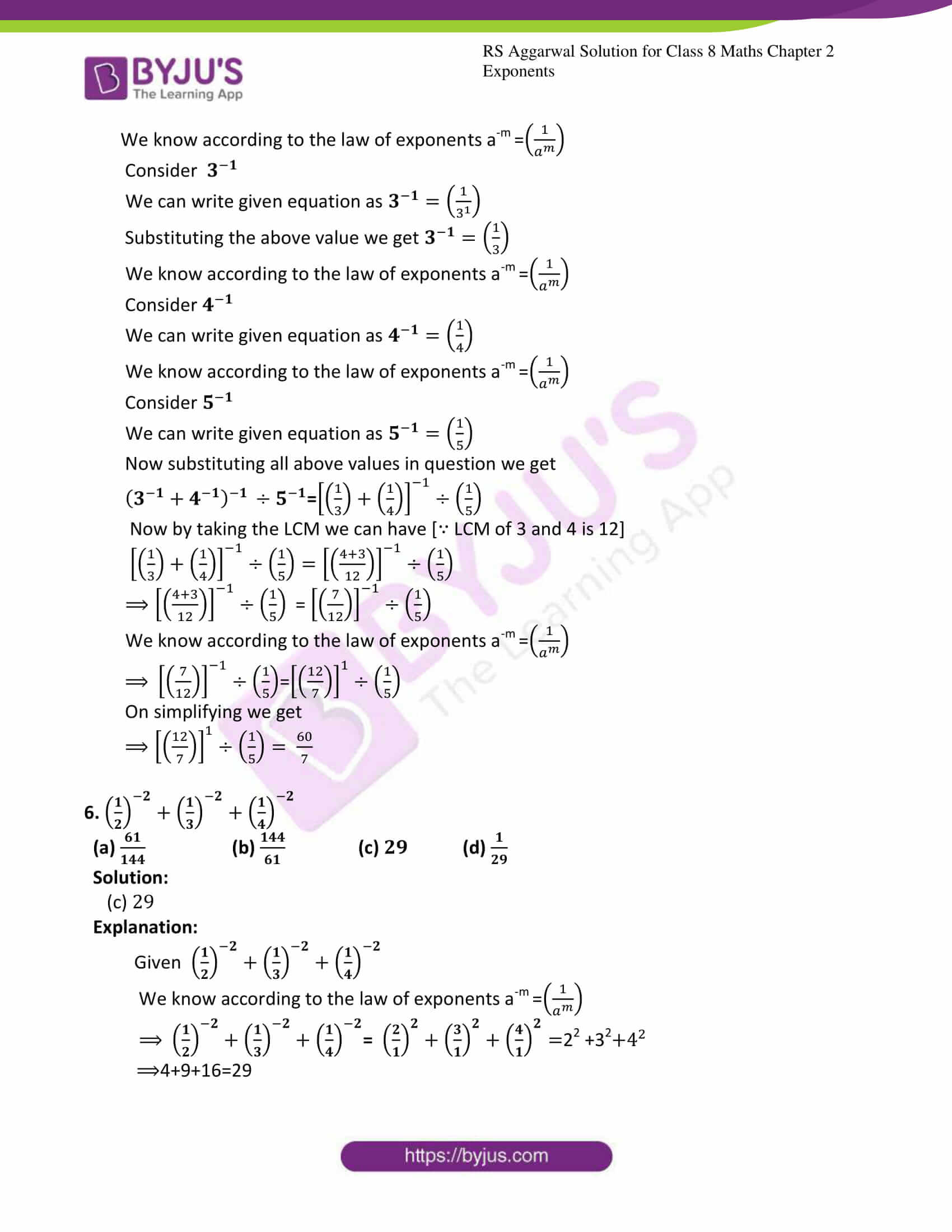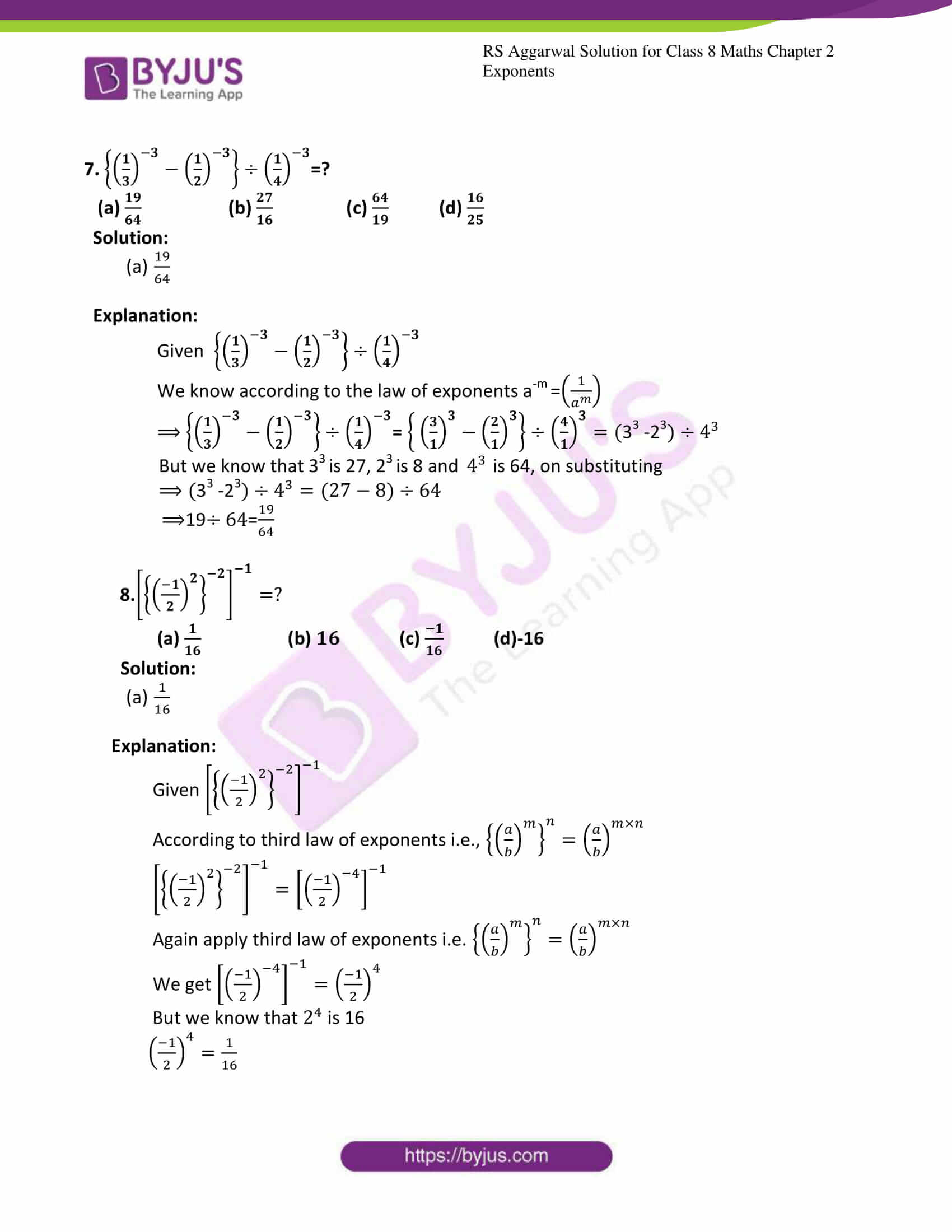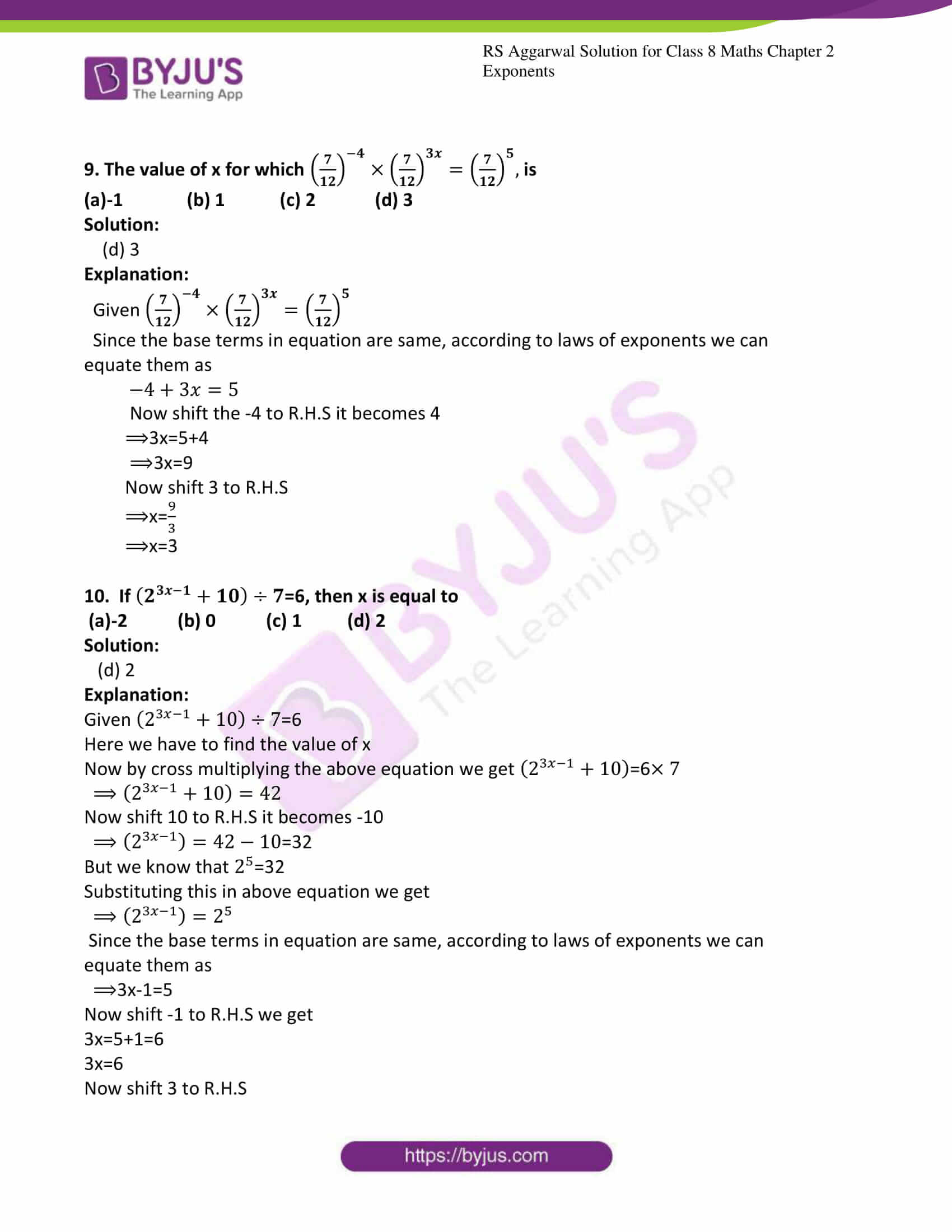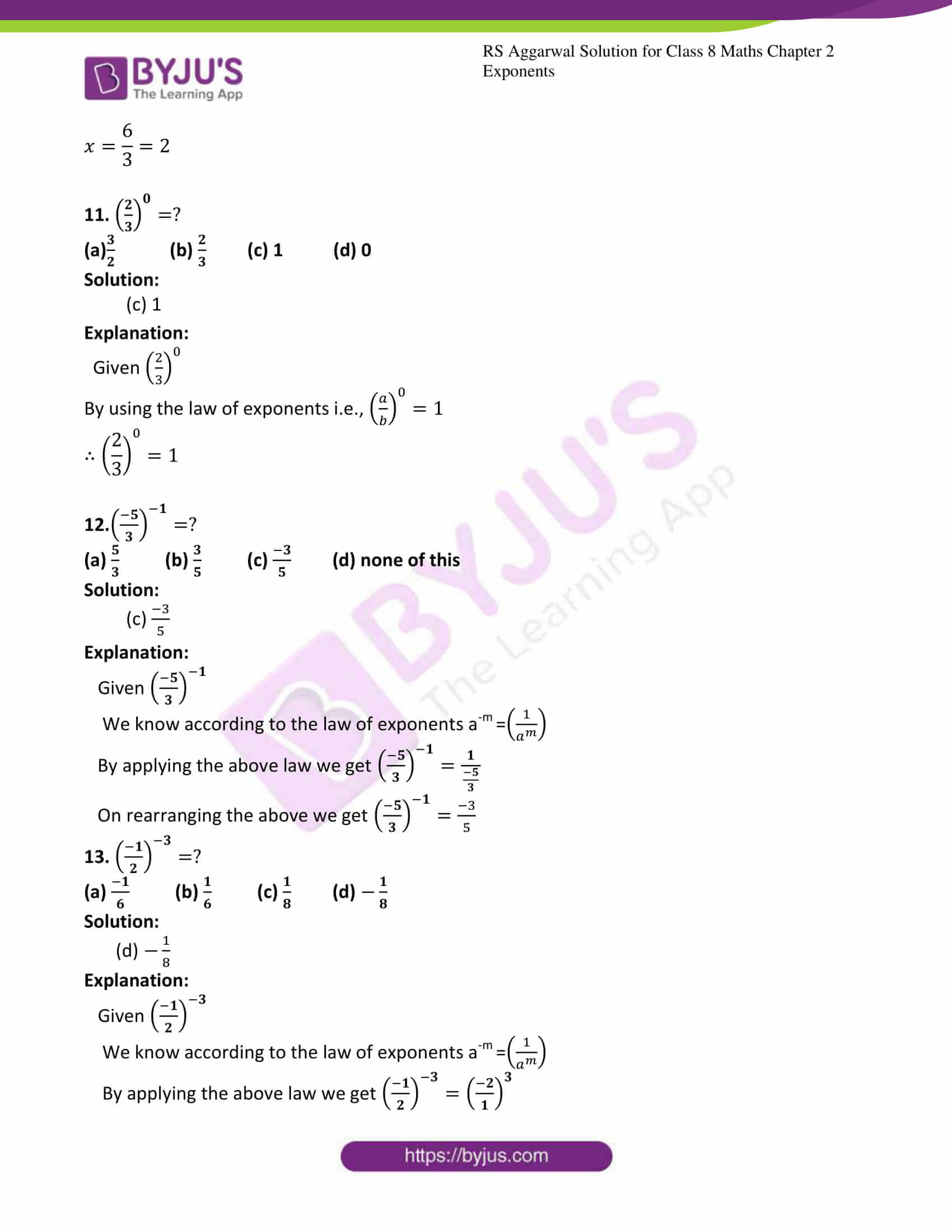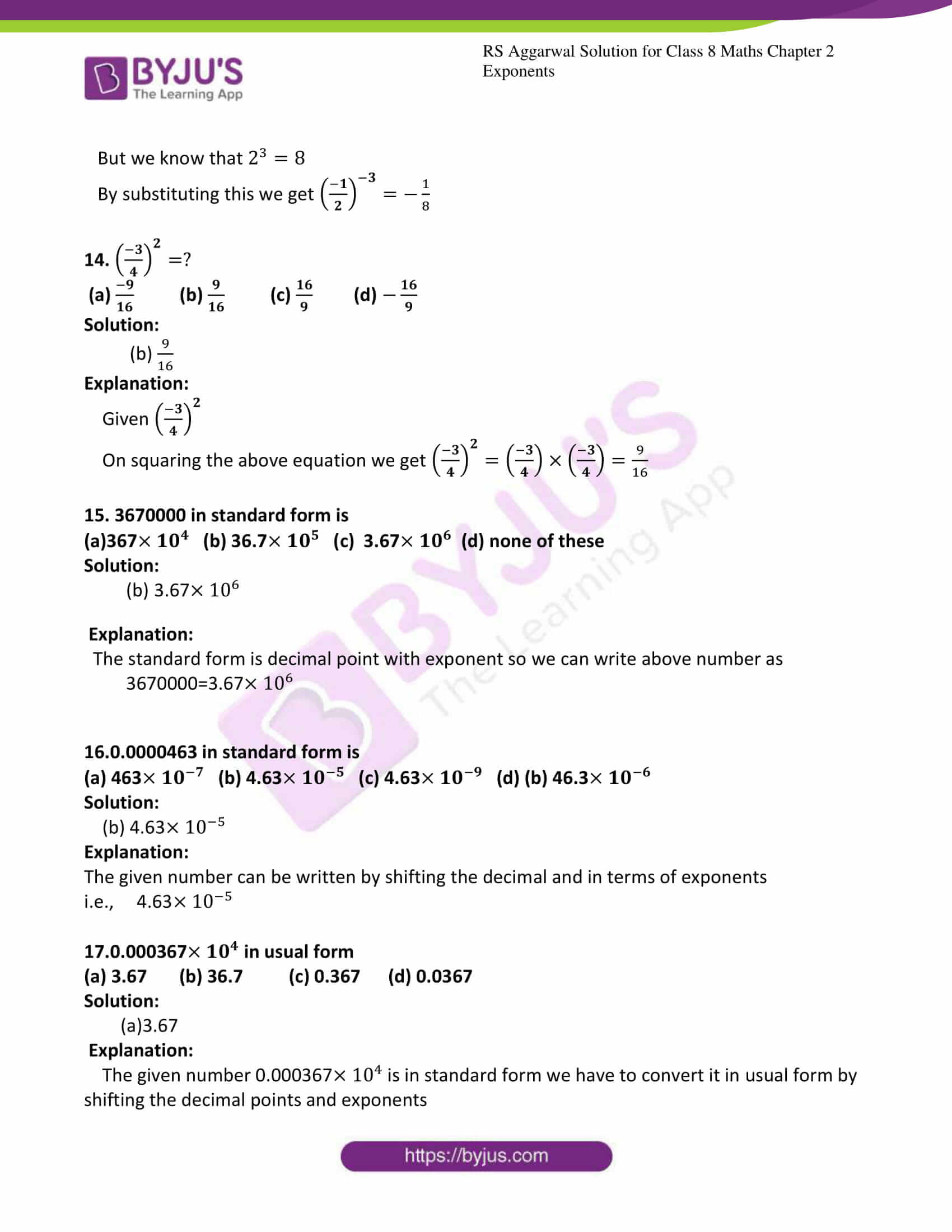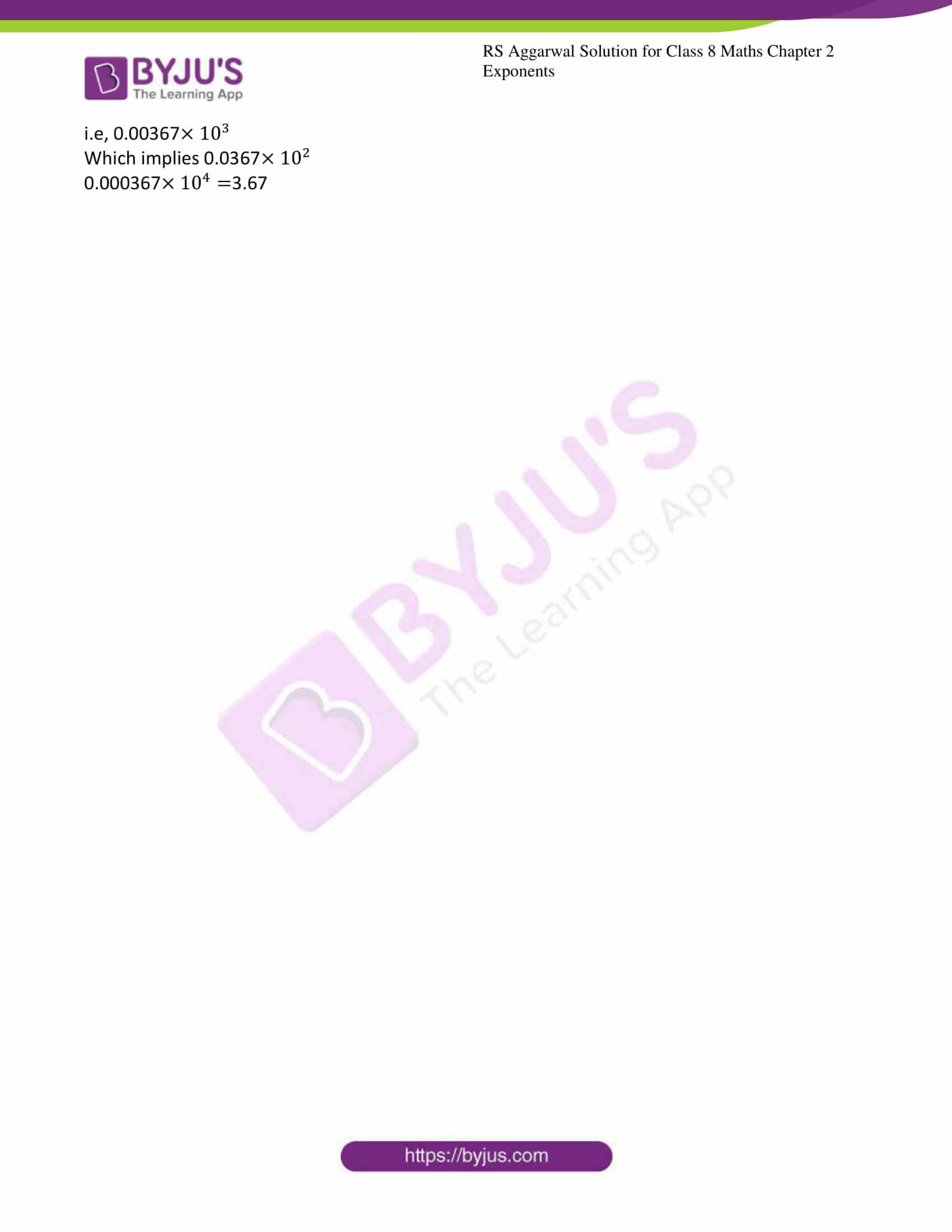### Exercise 2A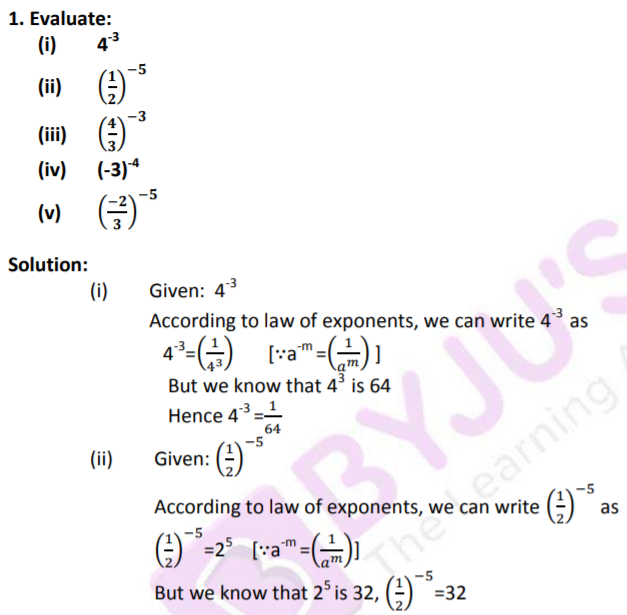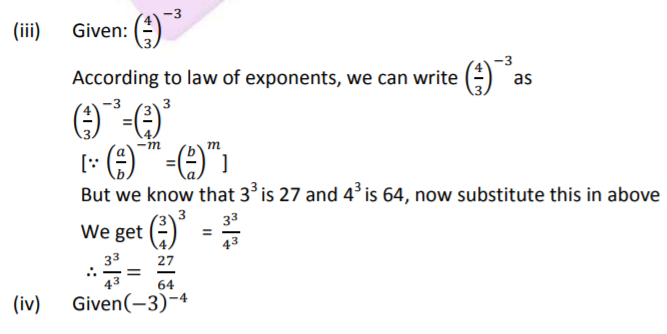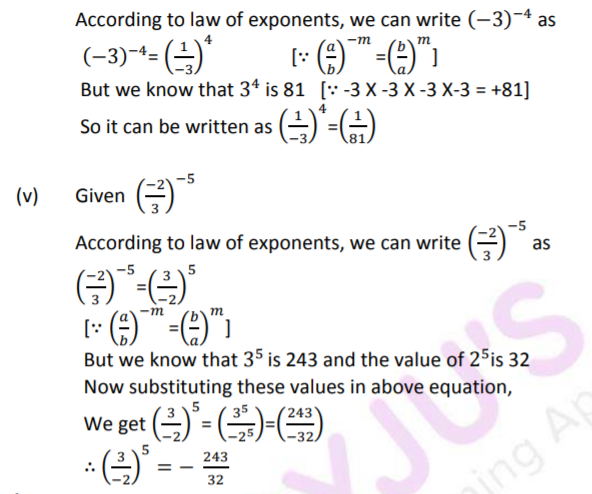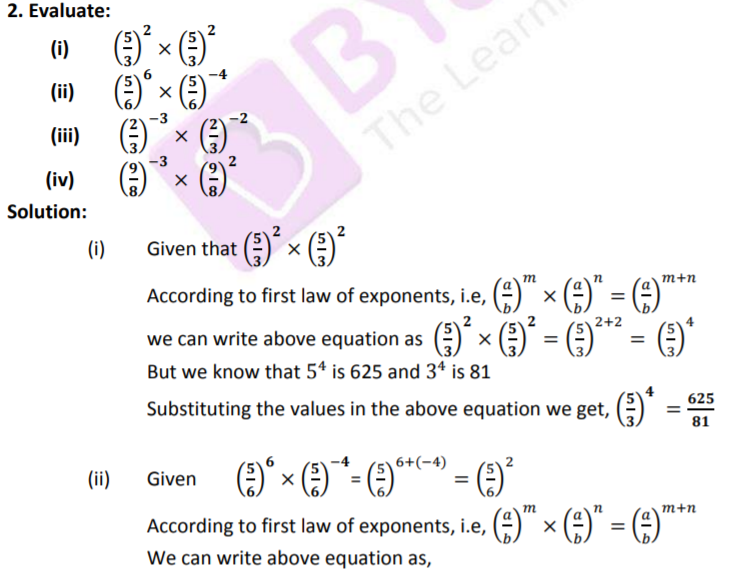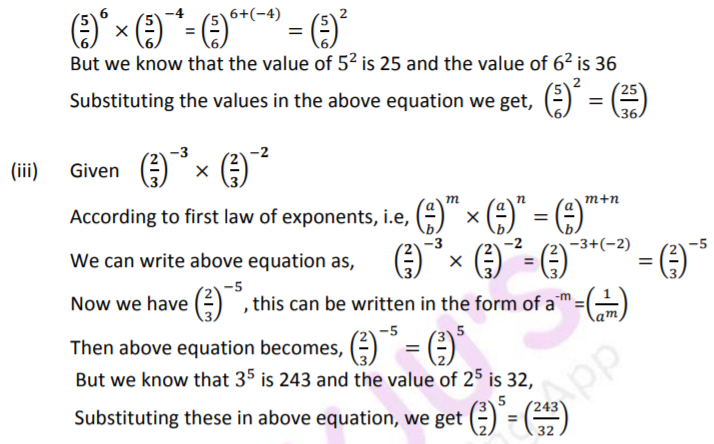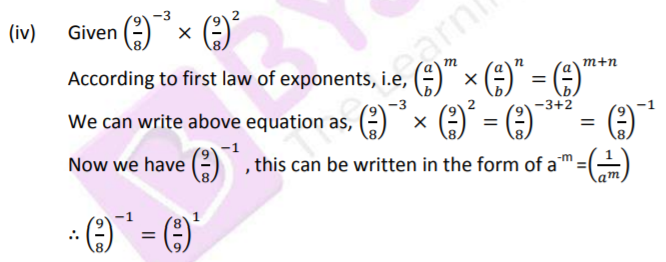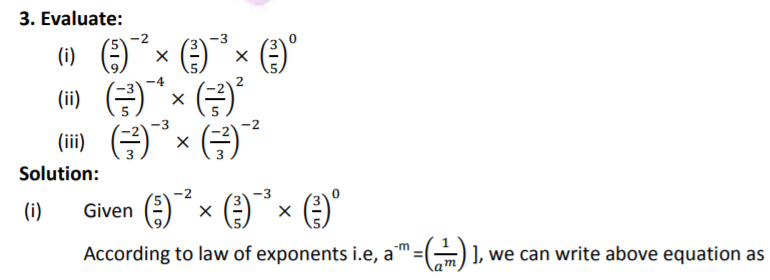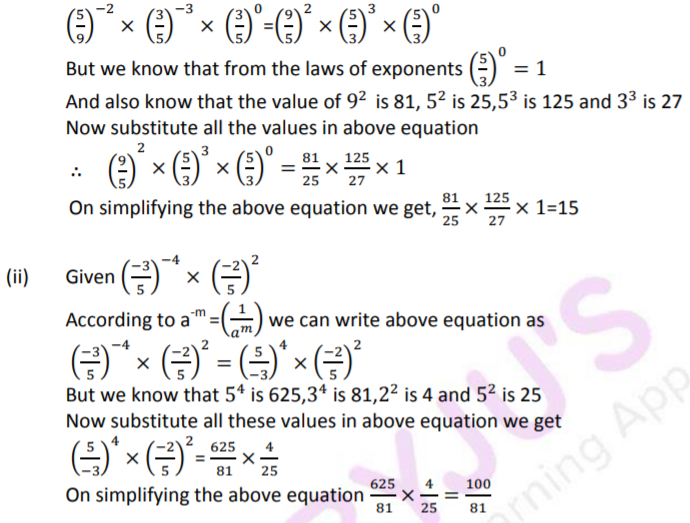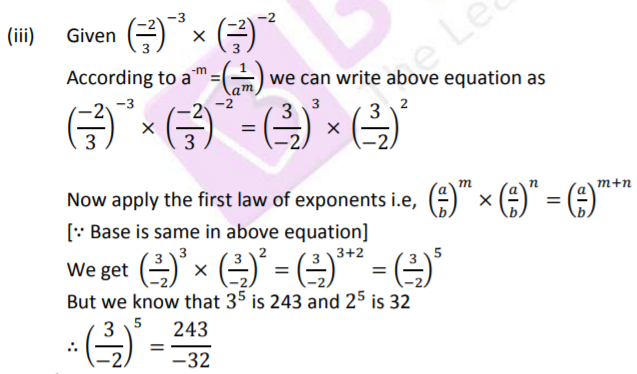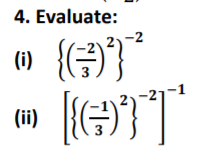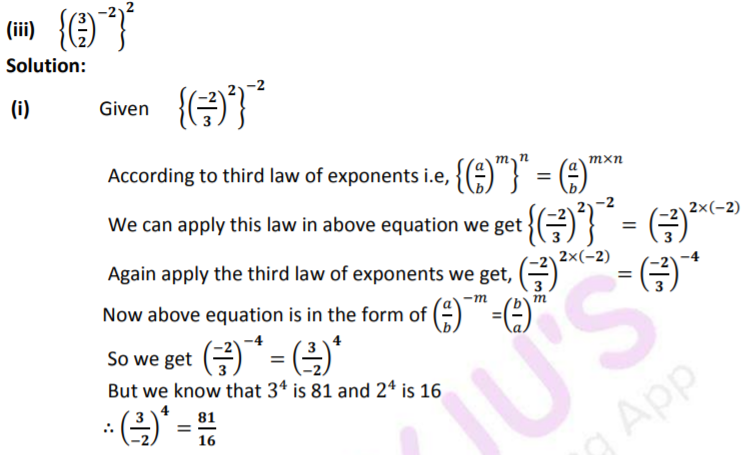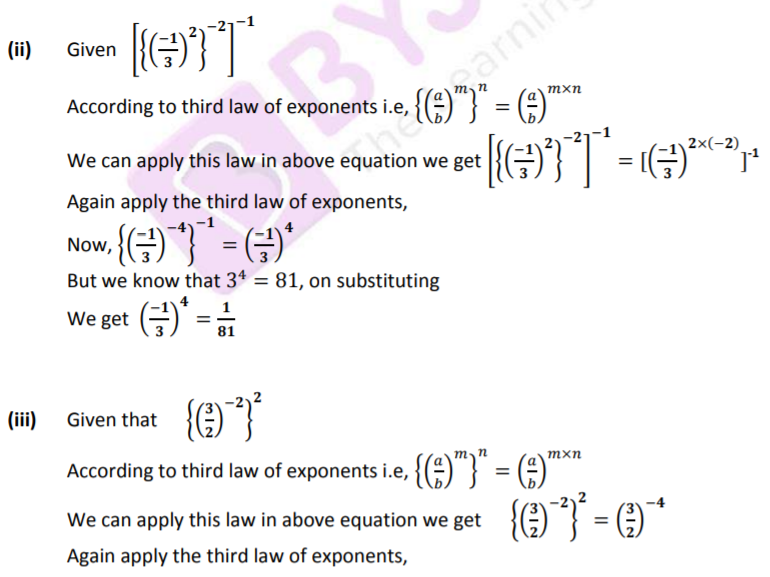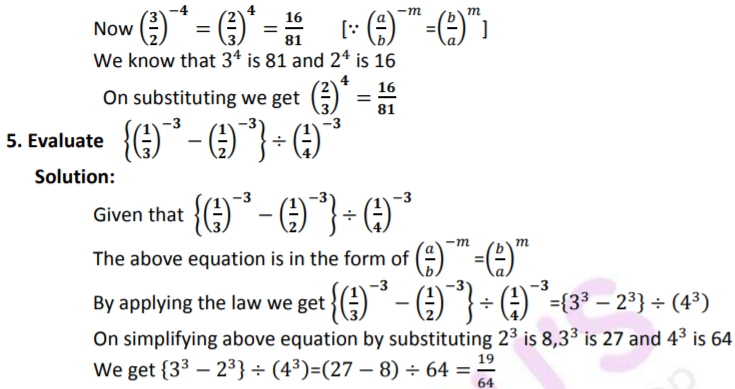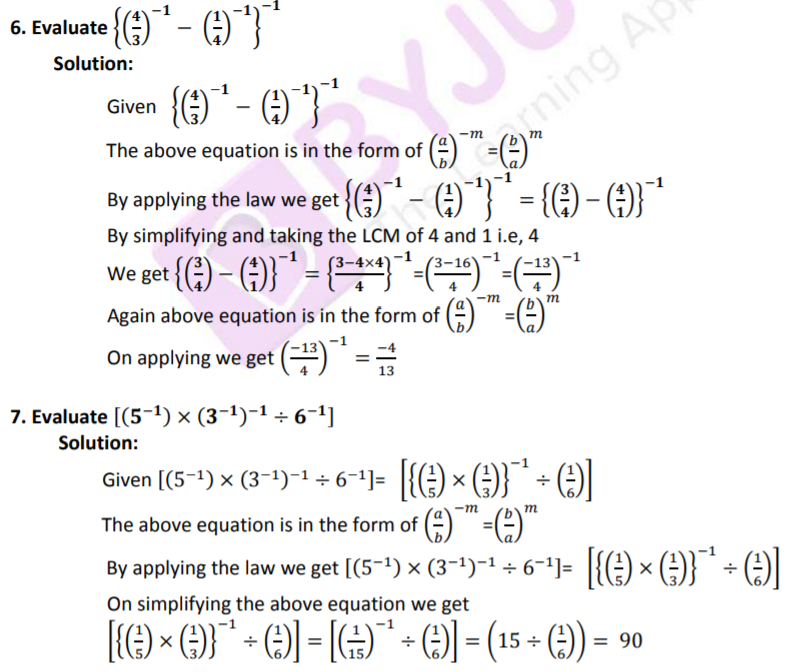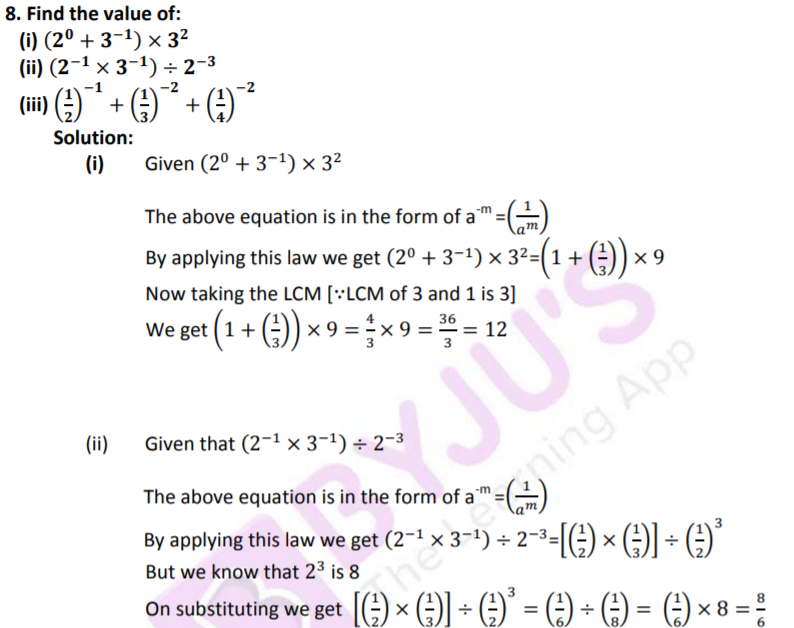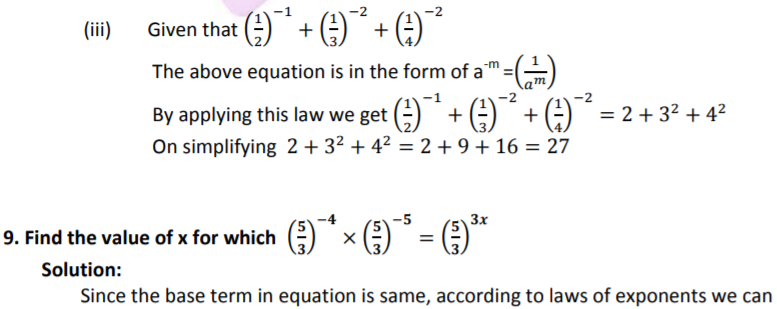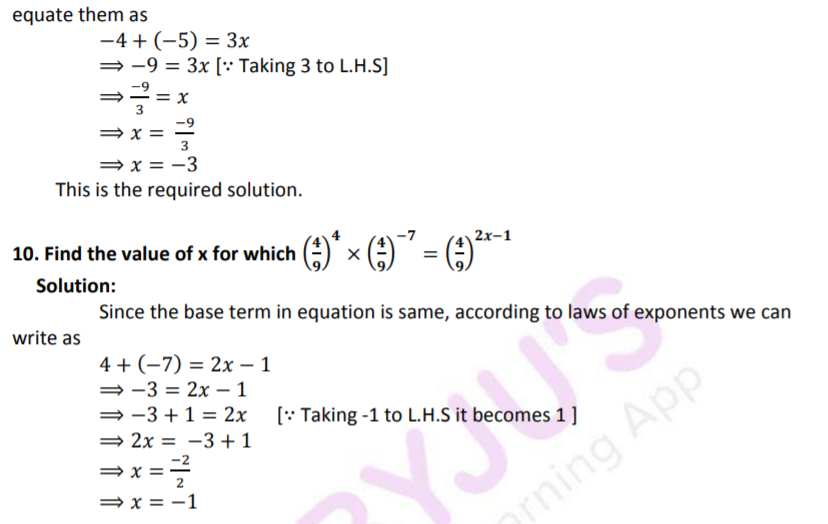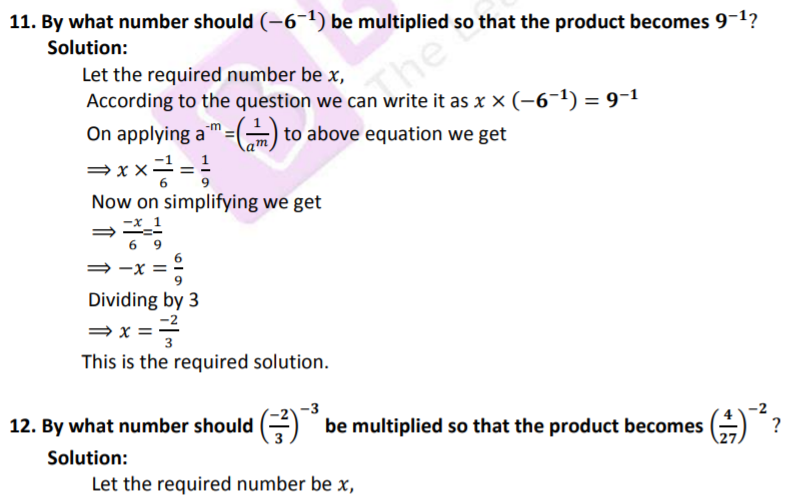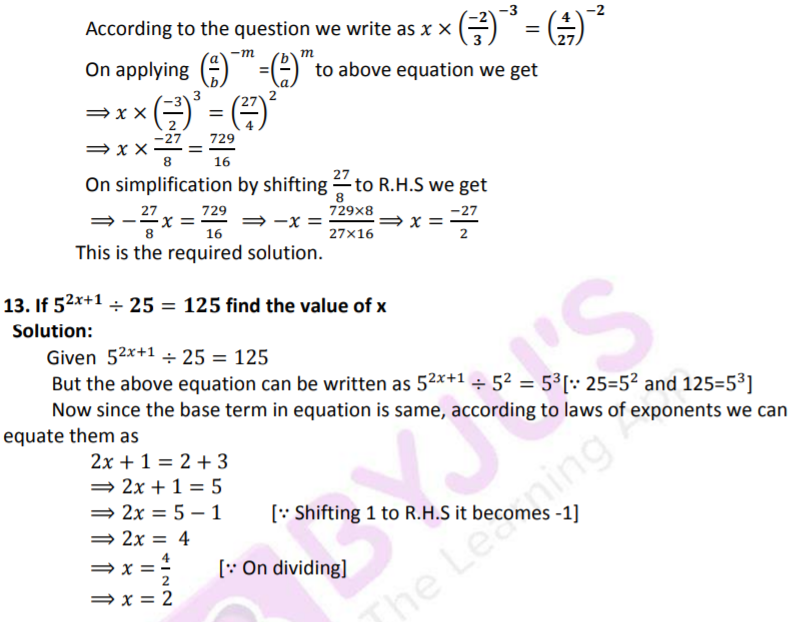### EXERCISE 2B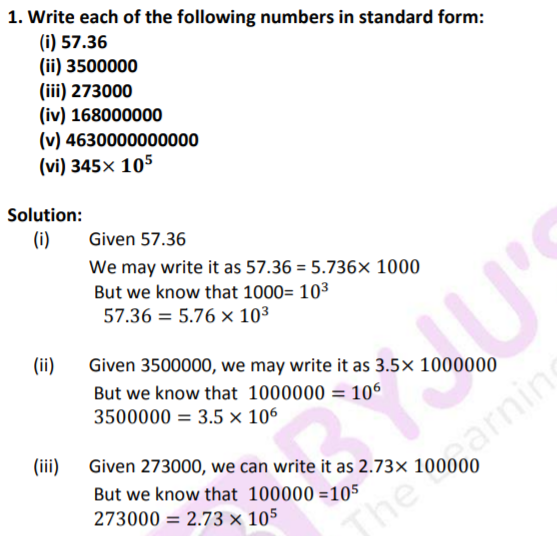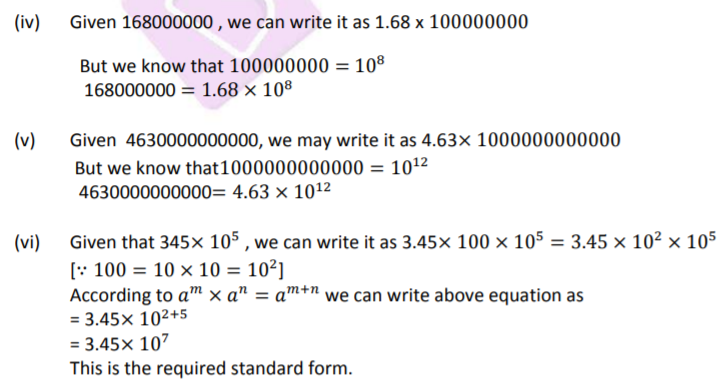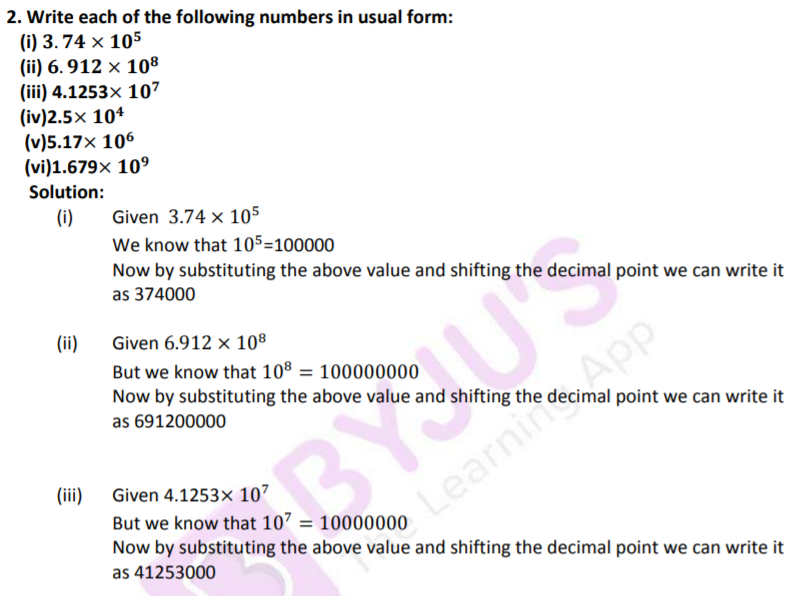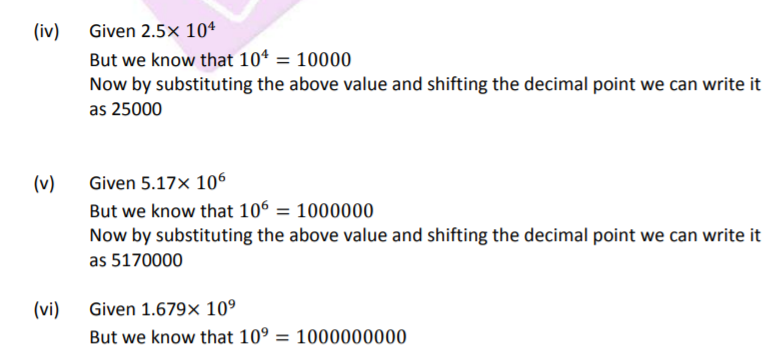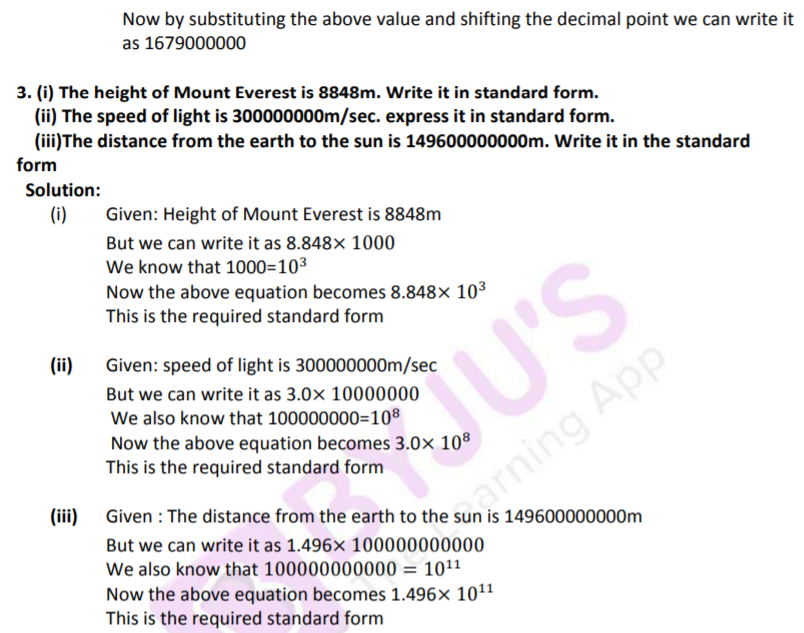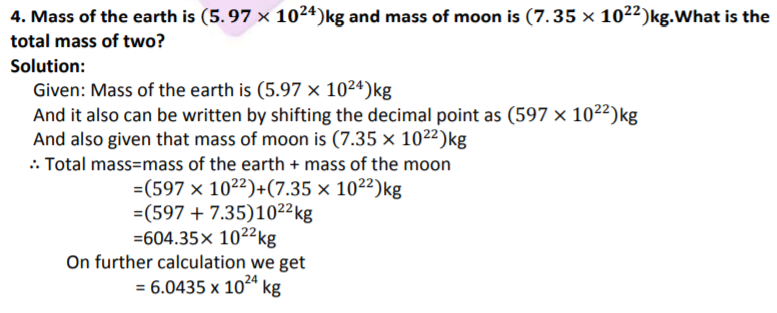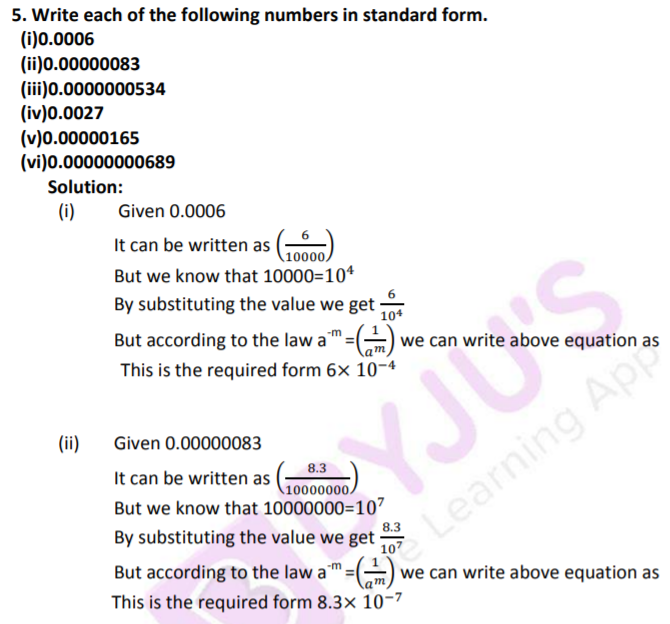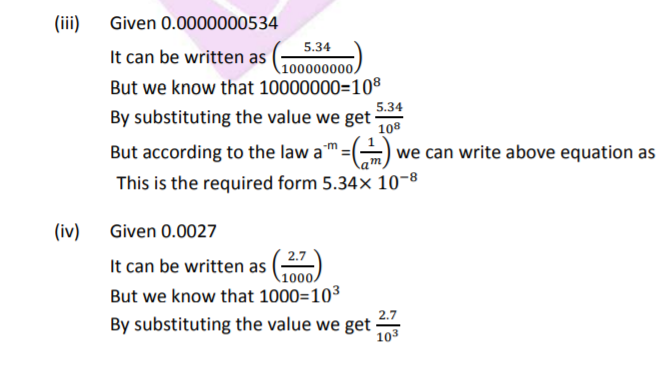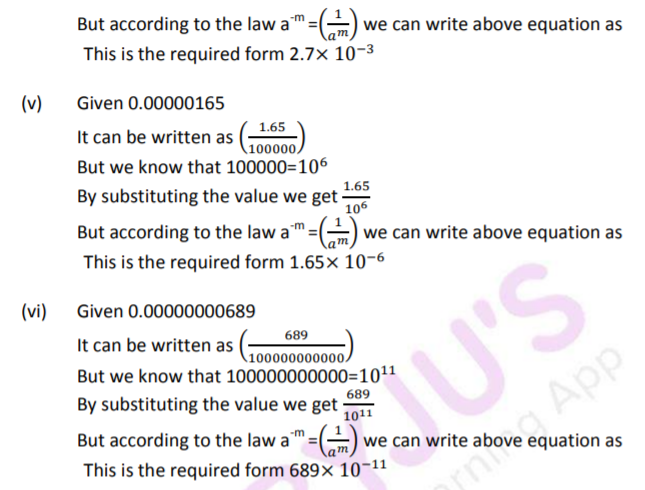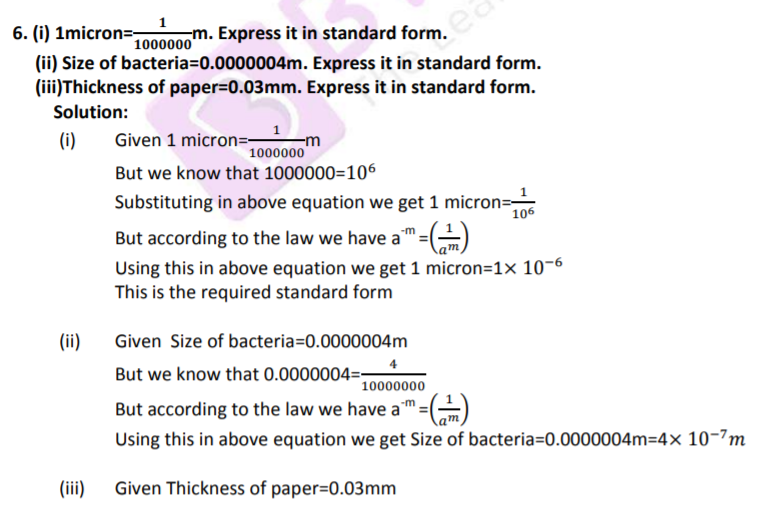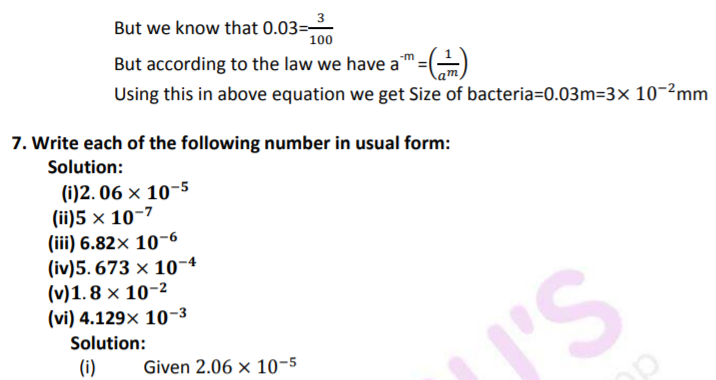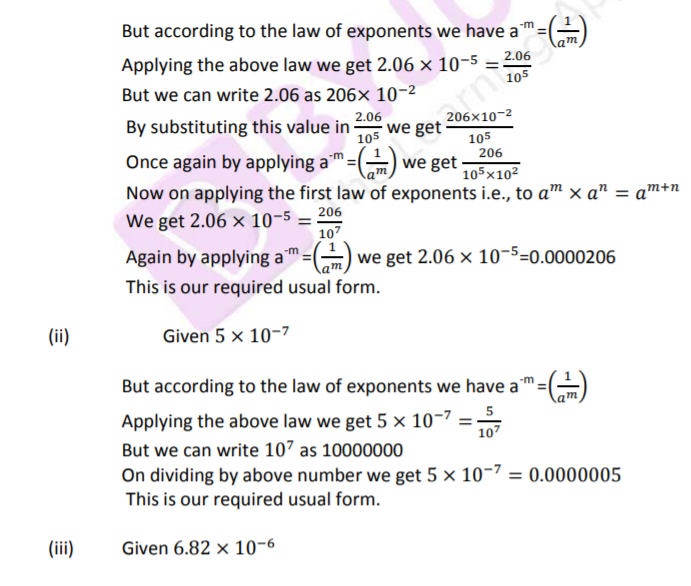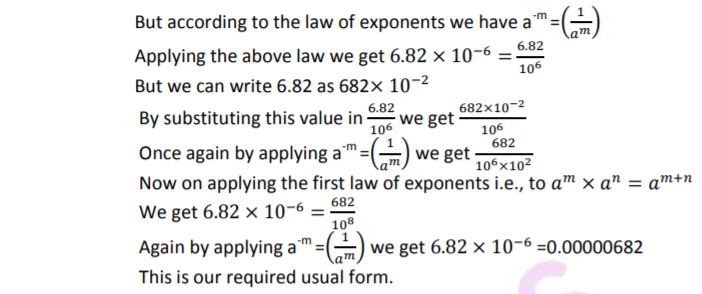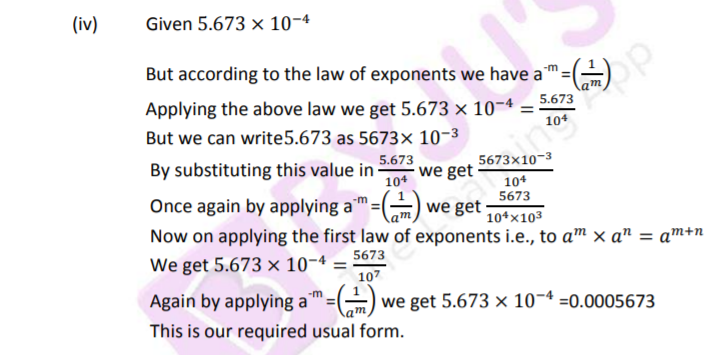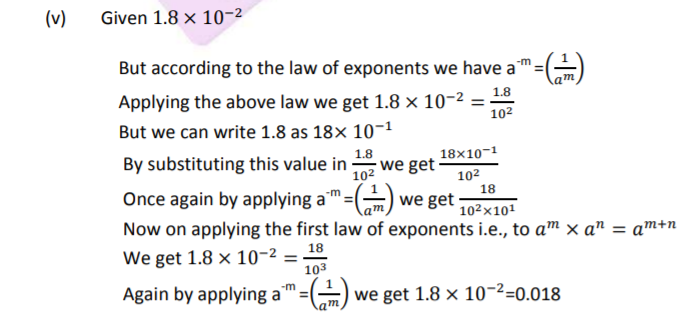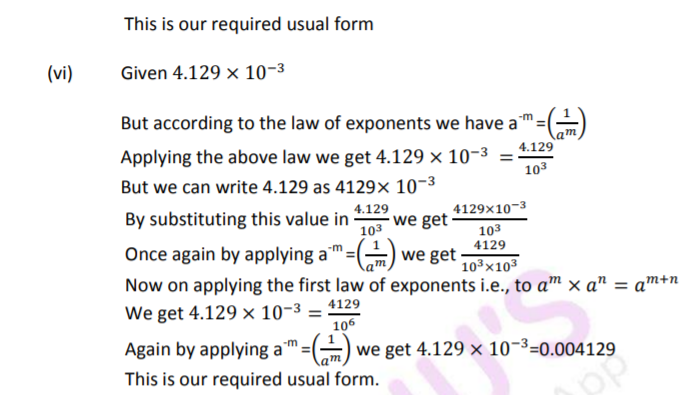### Exercise 2C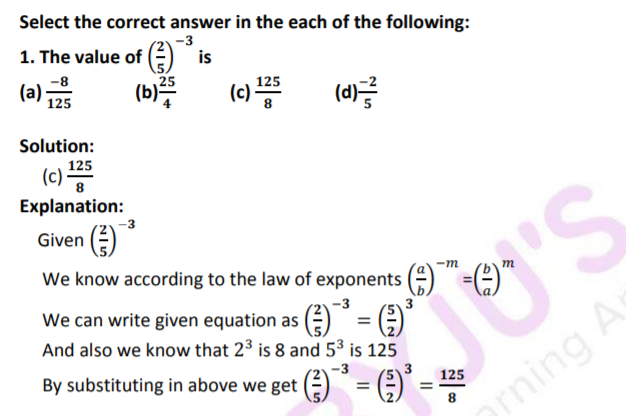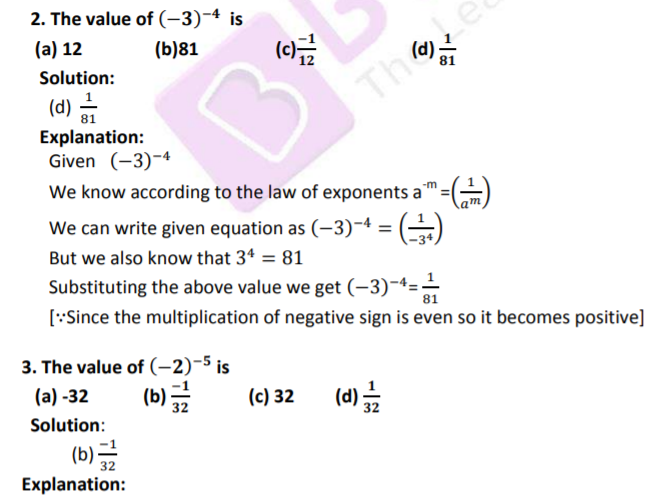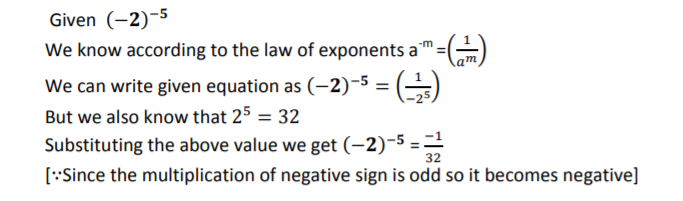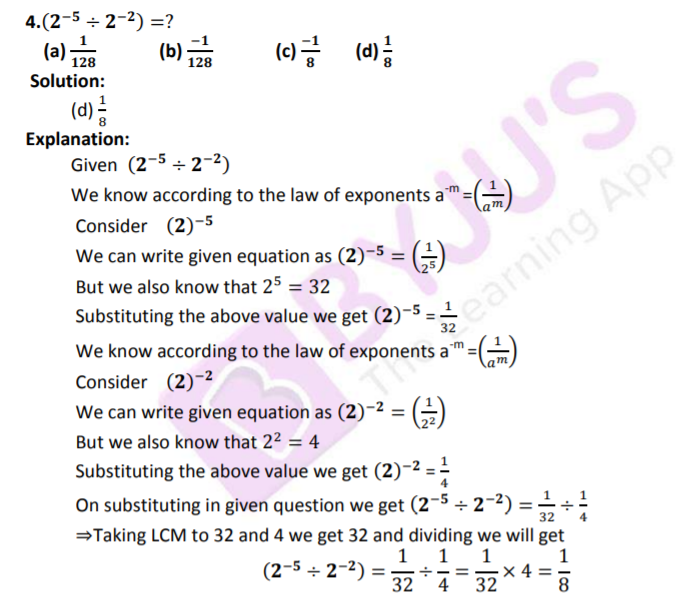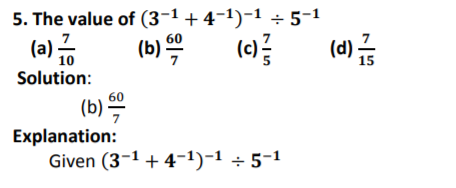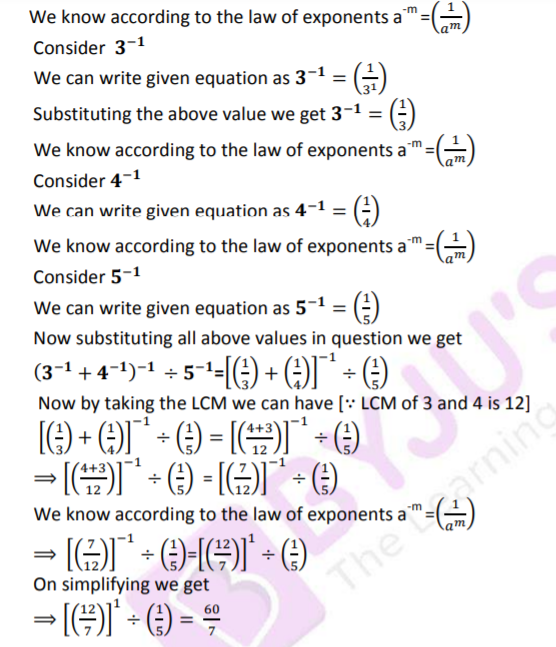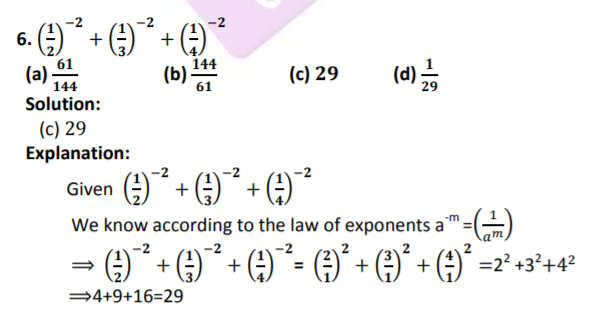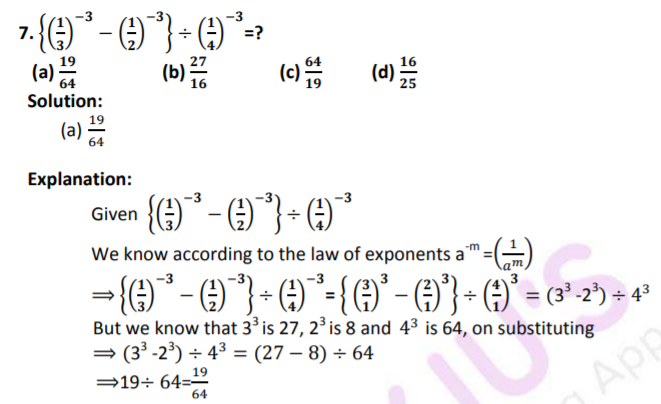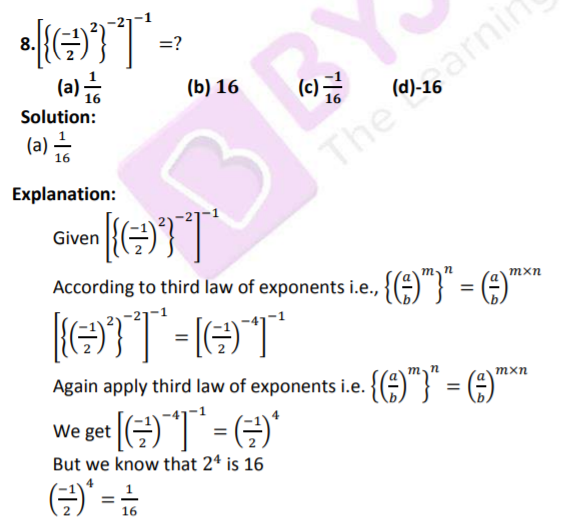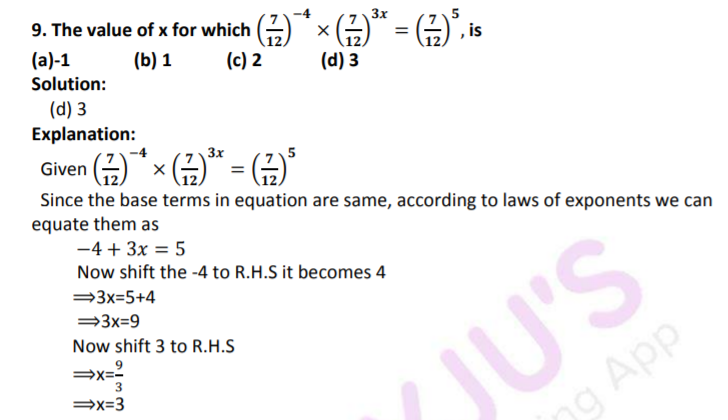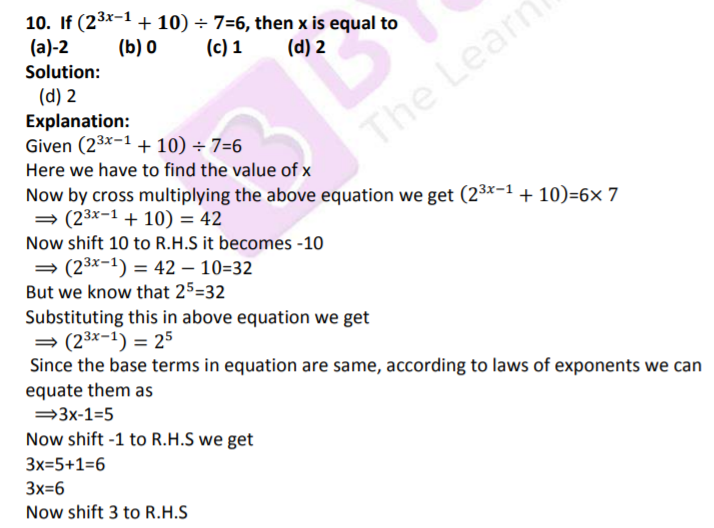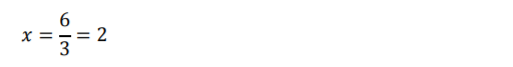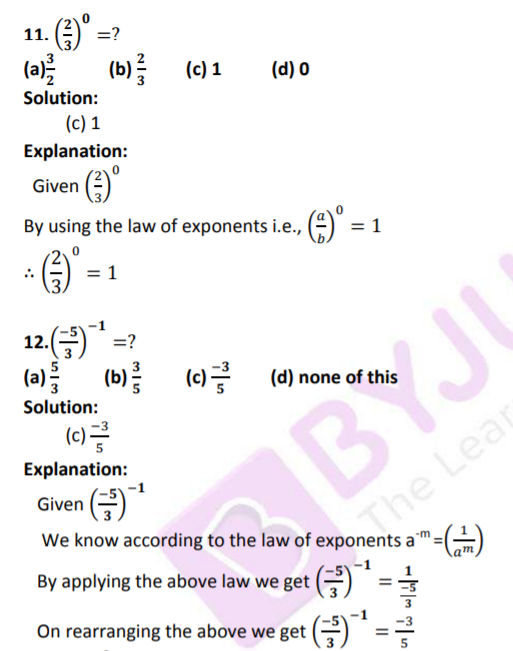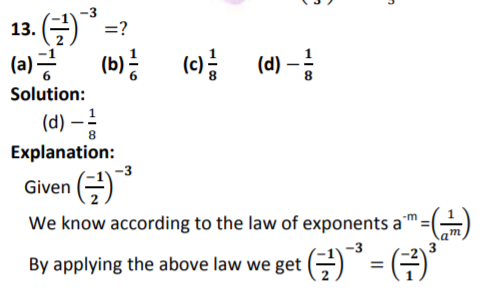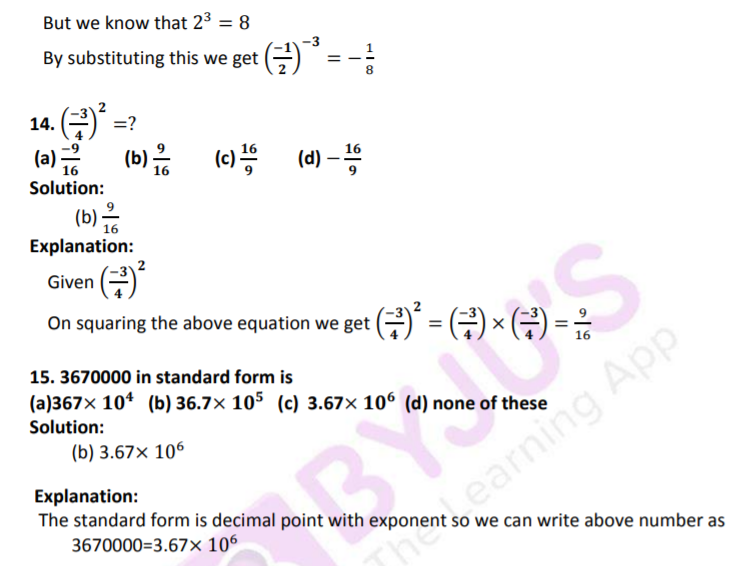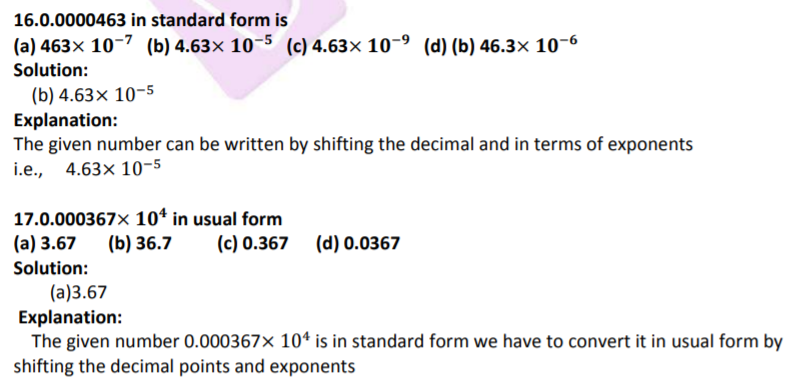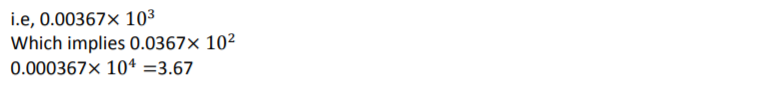## RS Aggarwal Solutions for Class 8 Maths Chapter 2- Exponents

Chapter 2, Exponents, contains 3 Exercises. RS Aggarwal Solutions for Class 8 Maths given here includes the answers to all the questions present in these exercises. Let us take a look at some of the concepts that are being discussed in this Chapter.

• Exponents of Rational number
• Positive Integral Exponent of a Rational number
• Negative Integral Exponent of a Rational number
• Laws of Exponents
• Expressing very large numbers in standard form
• Expressing very small numbers in standard form

Exercise 2A

Exercise 2B

Exercise 2C

### Chapter Brief of RS Aggarwal Solutions for Class 8 Maths Chapter 2 – Exponents

The RS Aggarwal Solutions for Class 8 Maths Chapter 2 – Exponents are widely used in high-level Mathematics, allowing you to represent a number or variable that is multiplied by itself a specified number of times and also used to represent very large numbers as well as very small numbers in standard form.